# RD Sharma Solutions for Class 6 Maths Chapter 20: Mensuration

The first thing which comes to our mind when we think about plane figures is boundaries and their regions. In this Chapter, we learn about the concept of area and perimeter of plane figures. These two concepts are of great importance in our daily lives which helps to determine their measurements.

The problems in this Chapter are based on shapes like closed and open curves, rectangle, square, equilateral triangle etc. These concepts are explained in a simple language based on the understanding capacity of students to make it simple for them during exam preparation. RD Sharma Solutions for Class 6 Chapter 20 Mensuration PDF are provided here.

## RD Sharma Solutions for Class 6 Maths Chapter 20: Mensuration Download PDF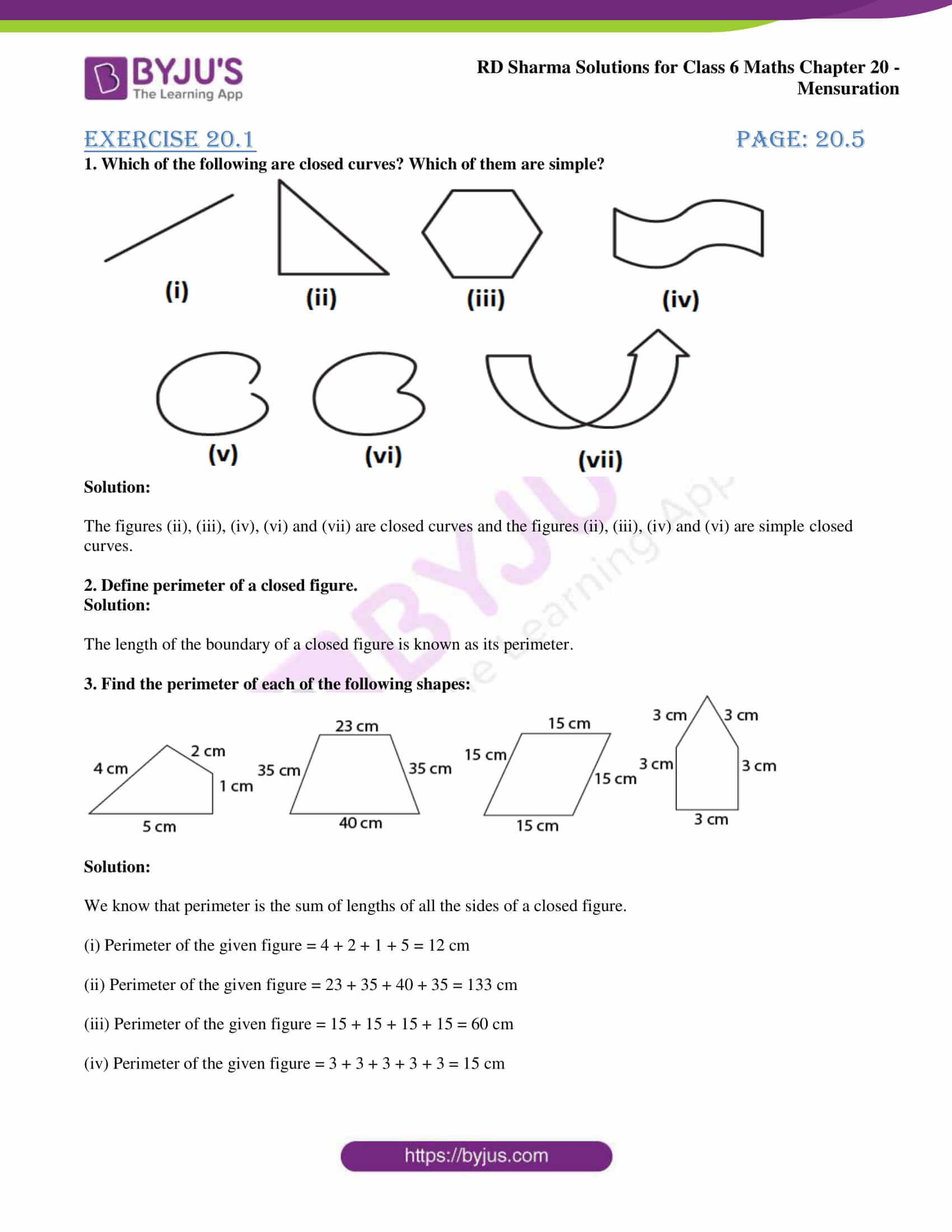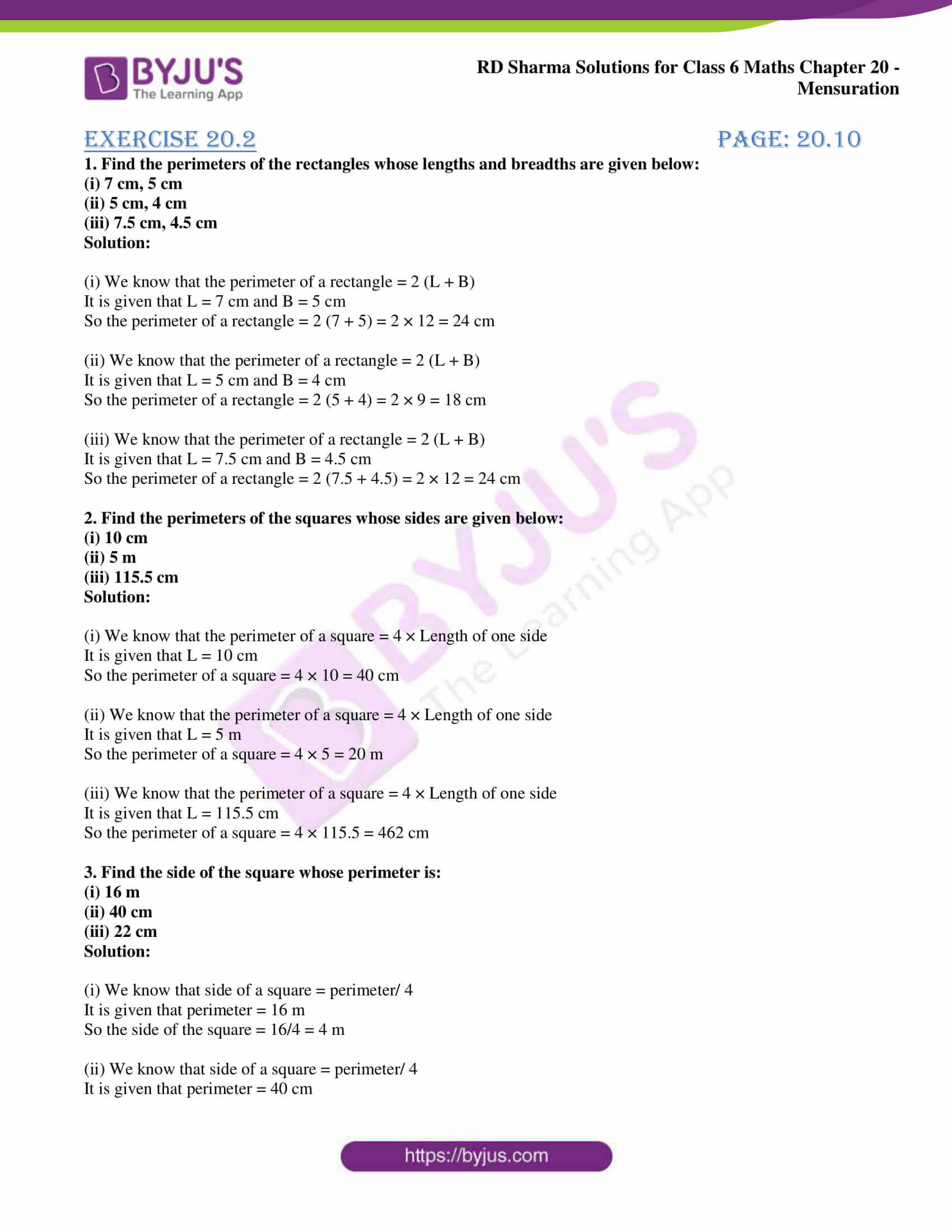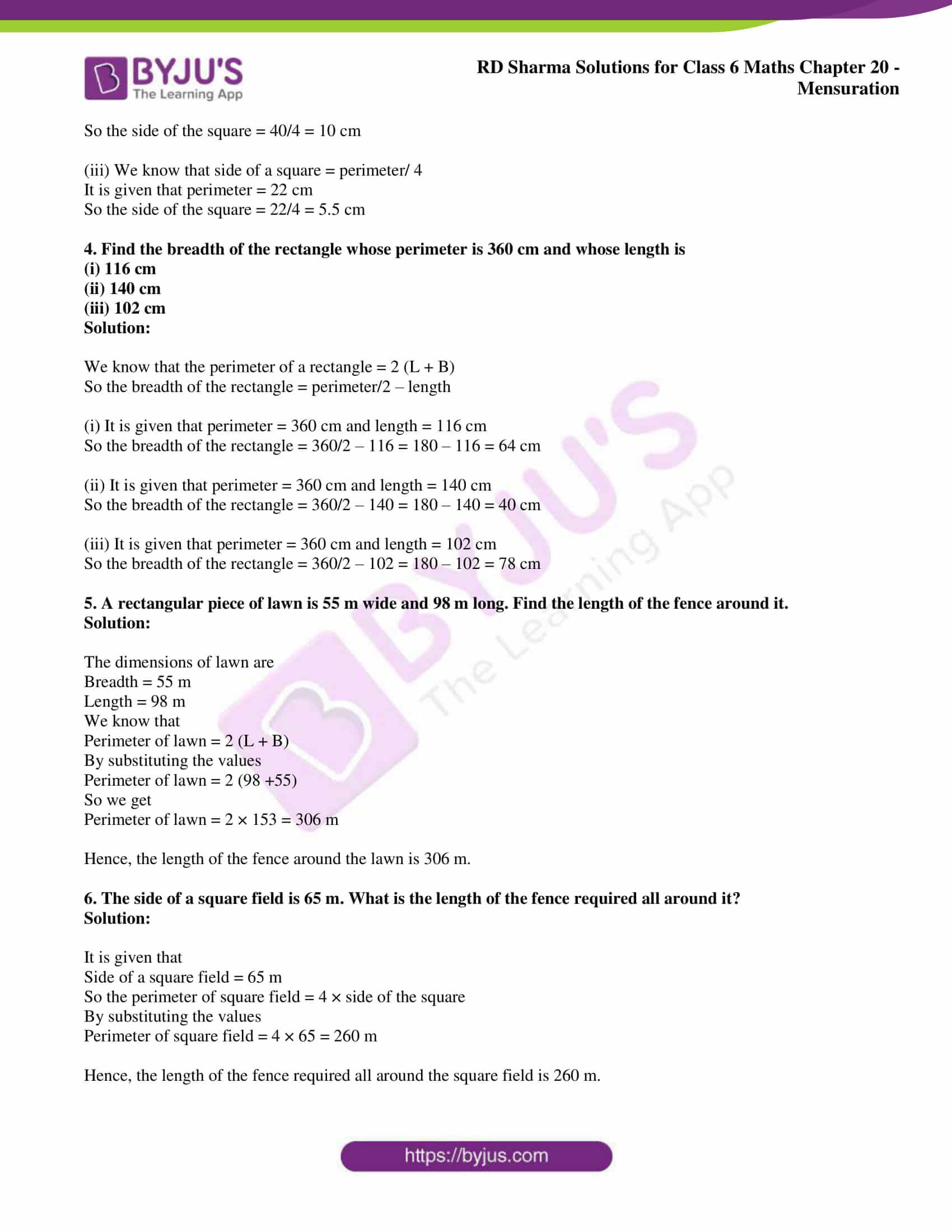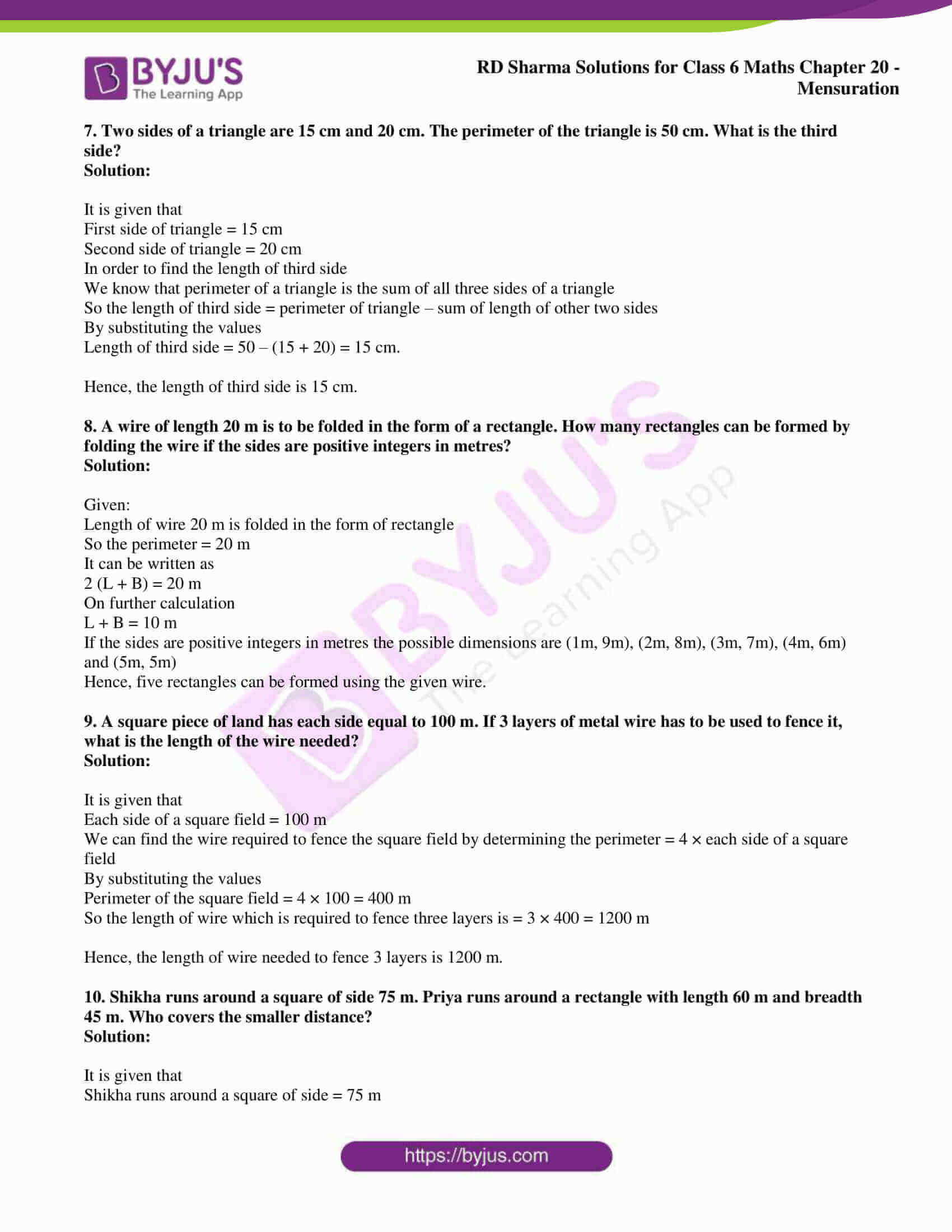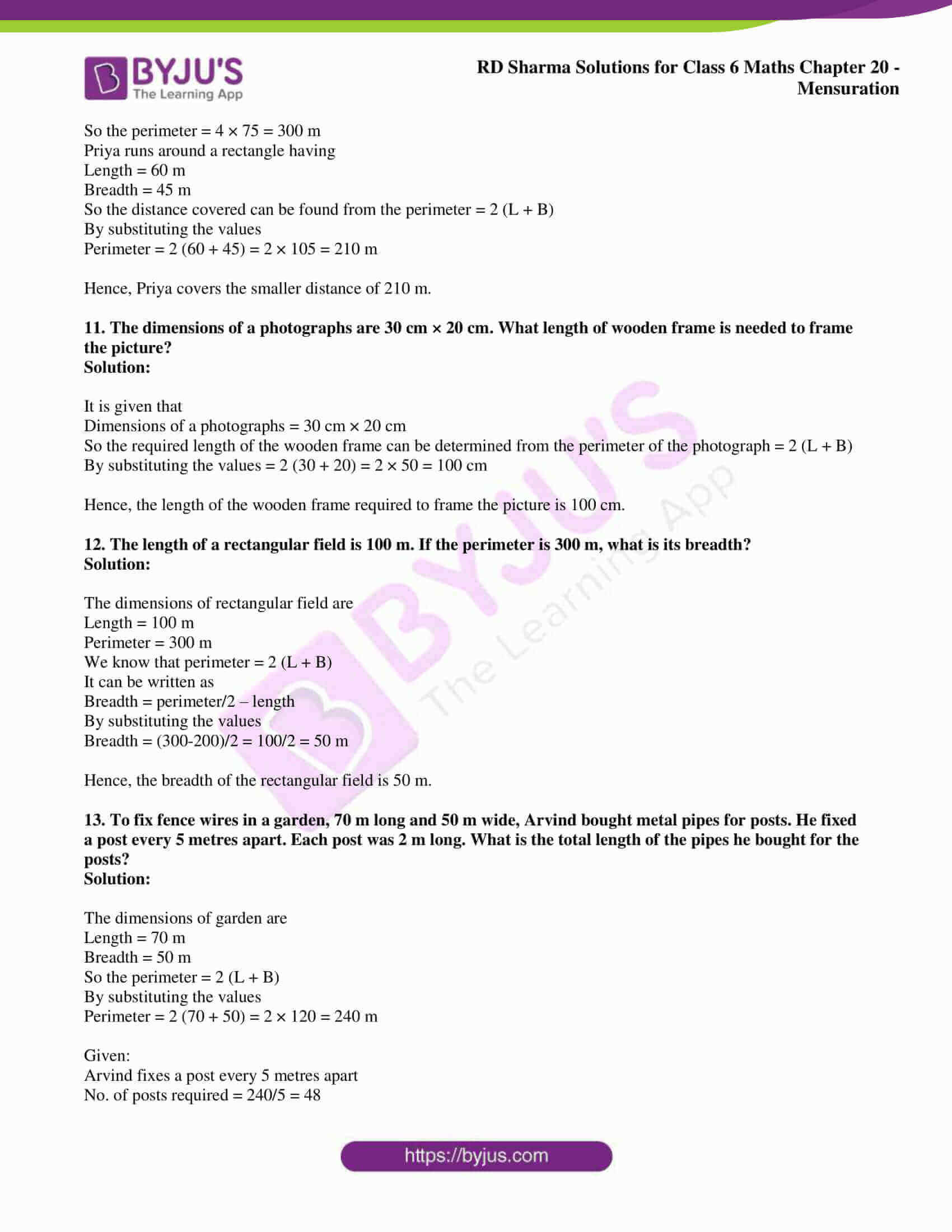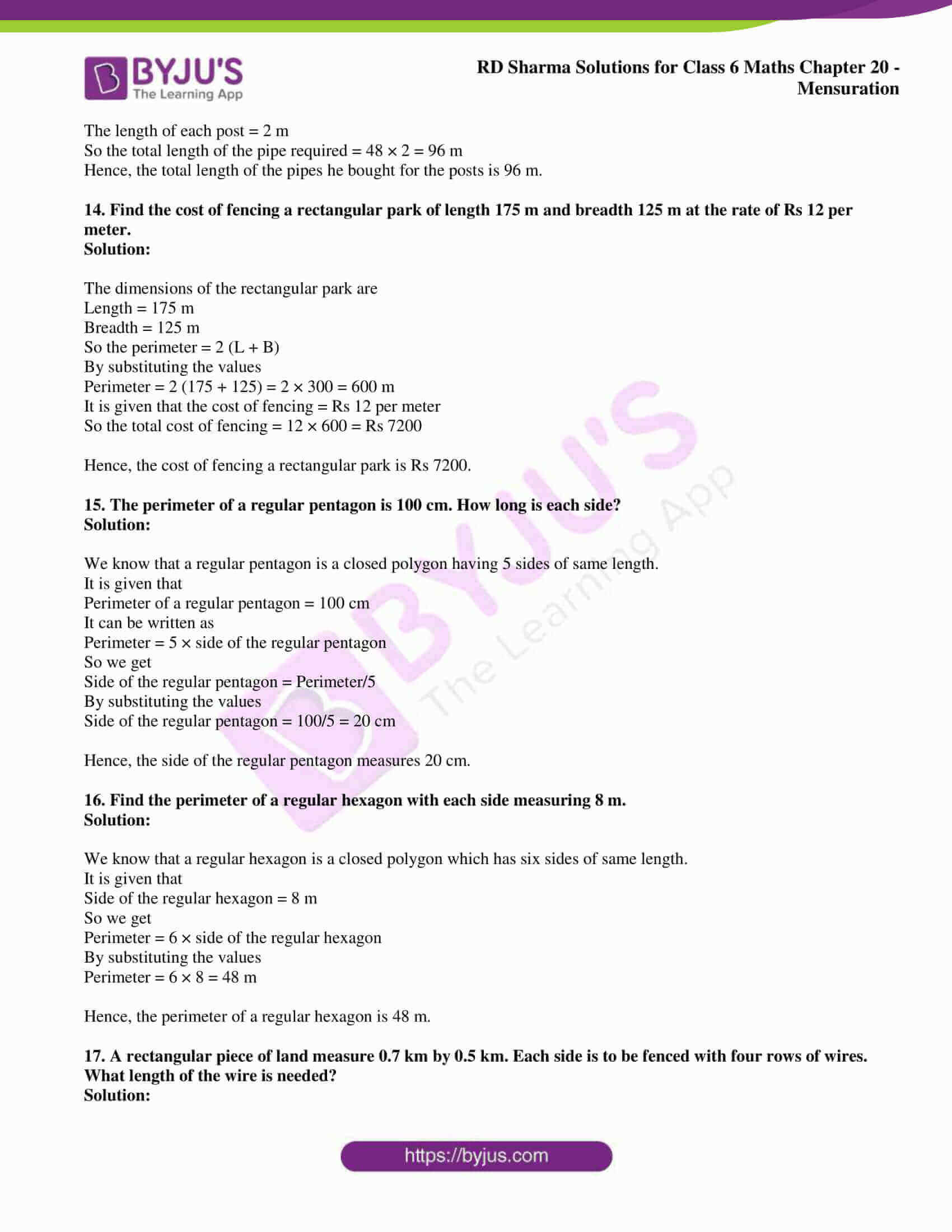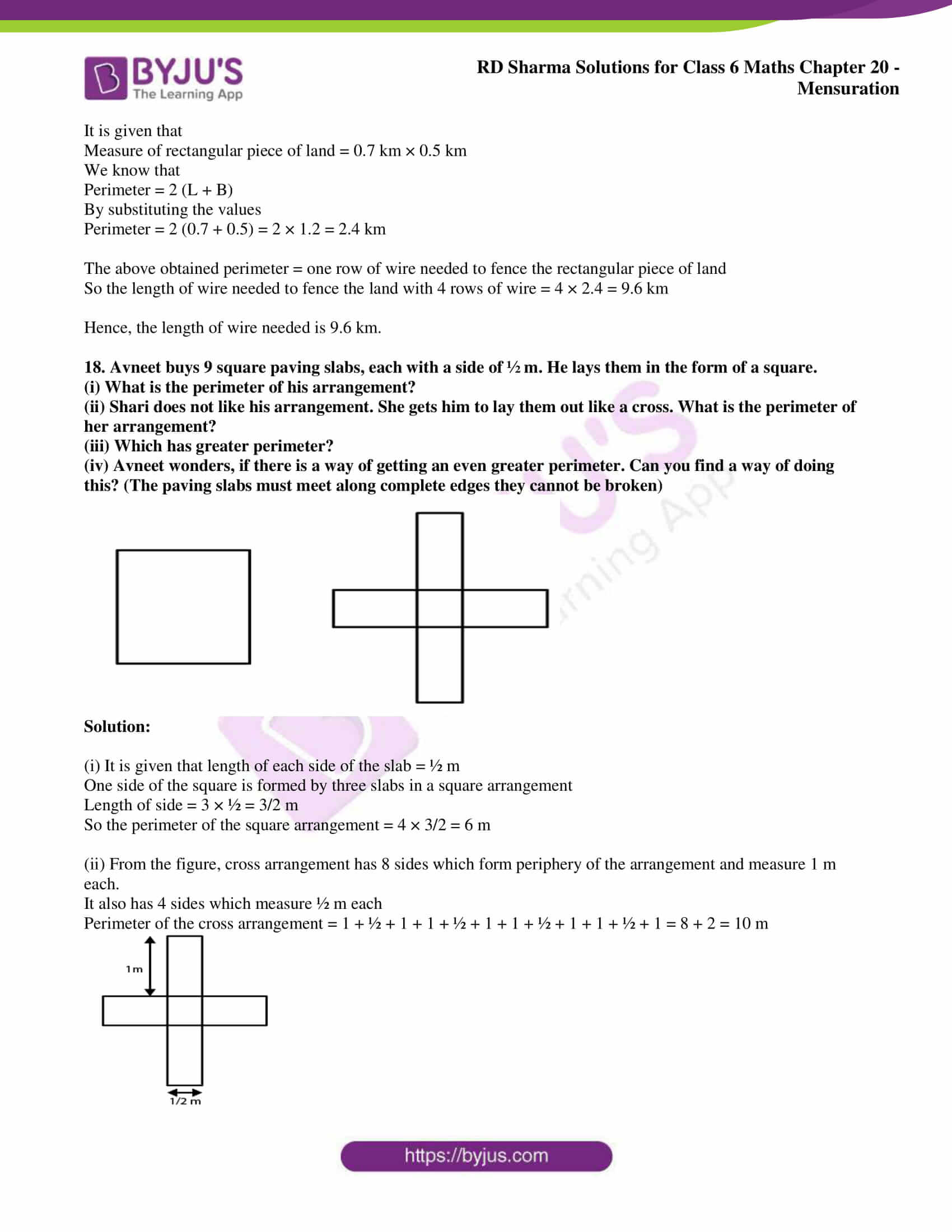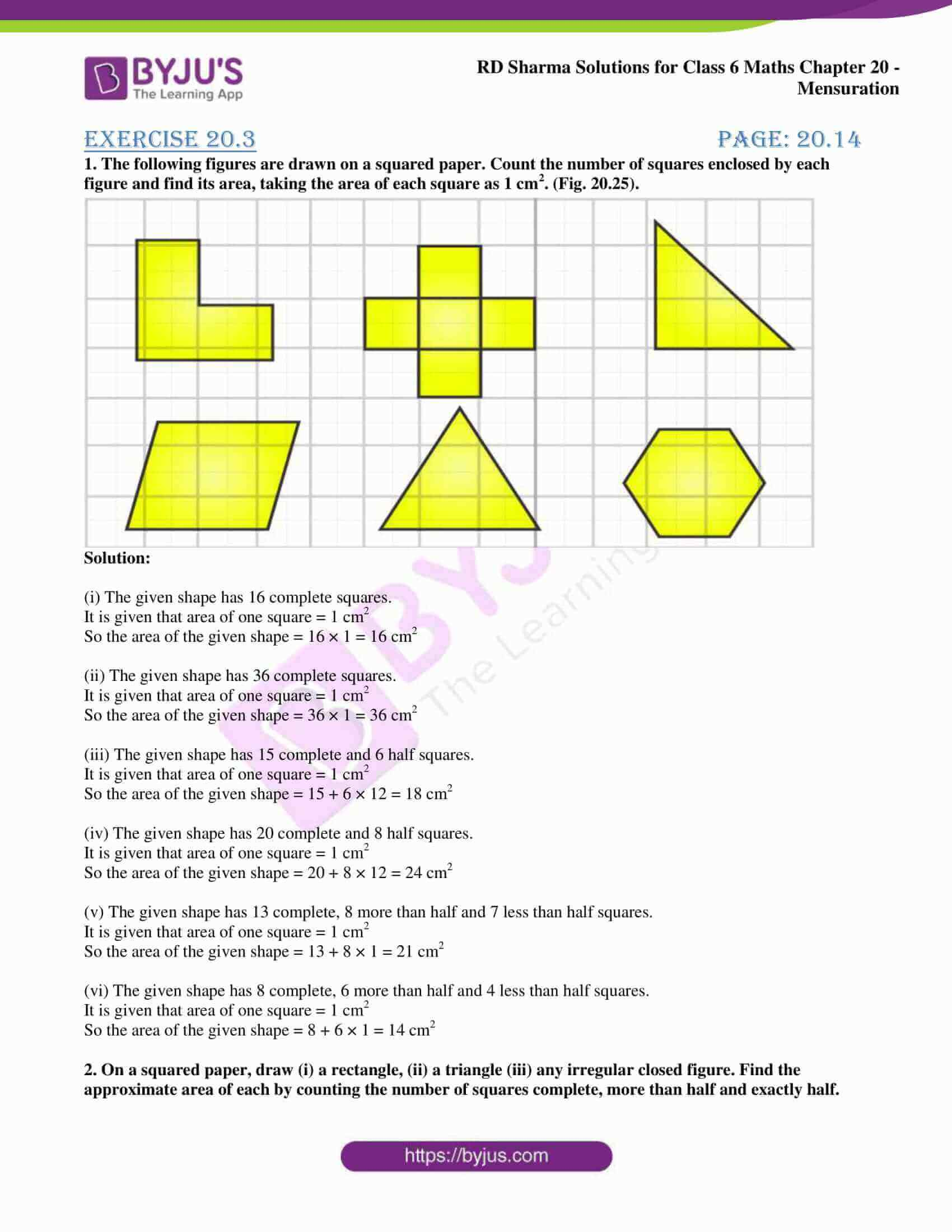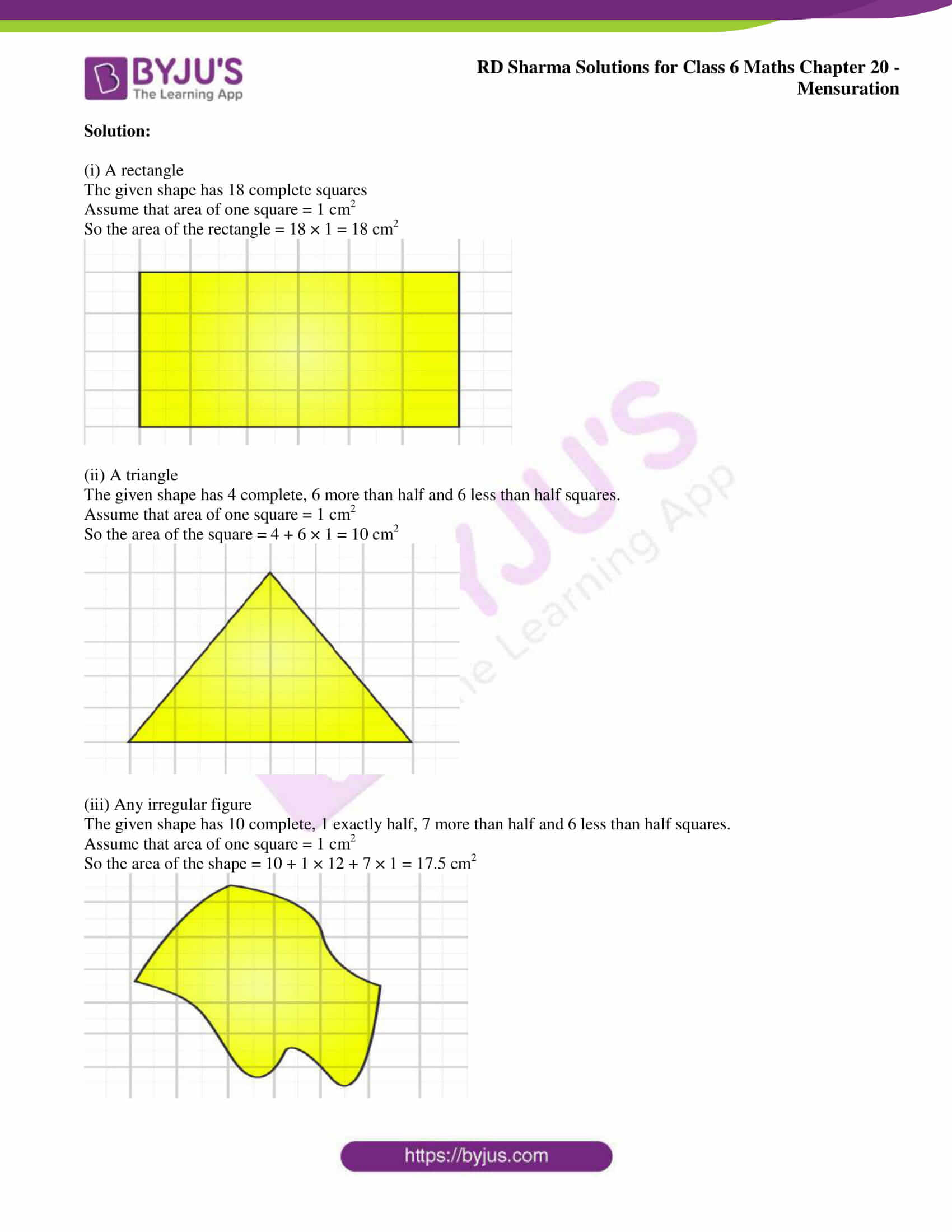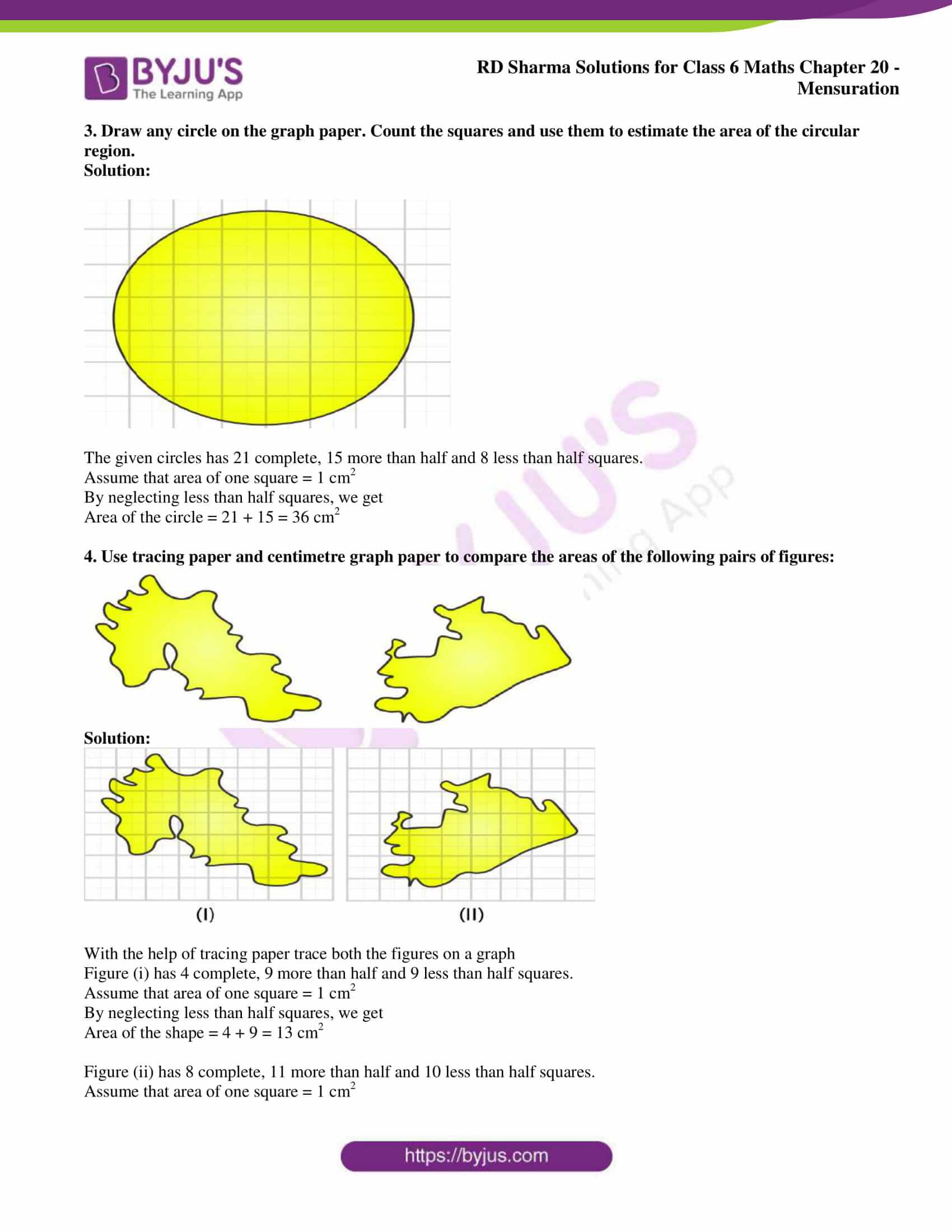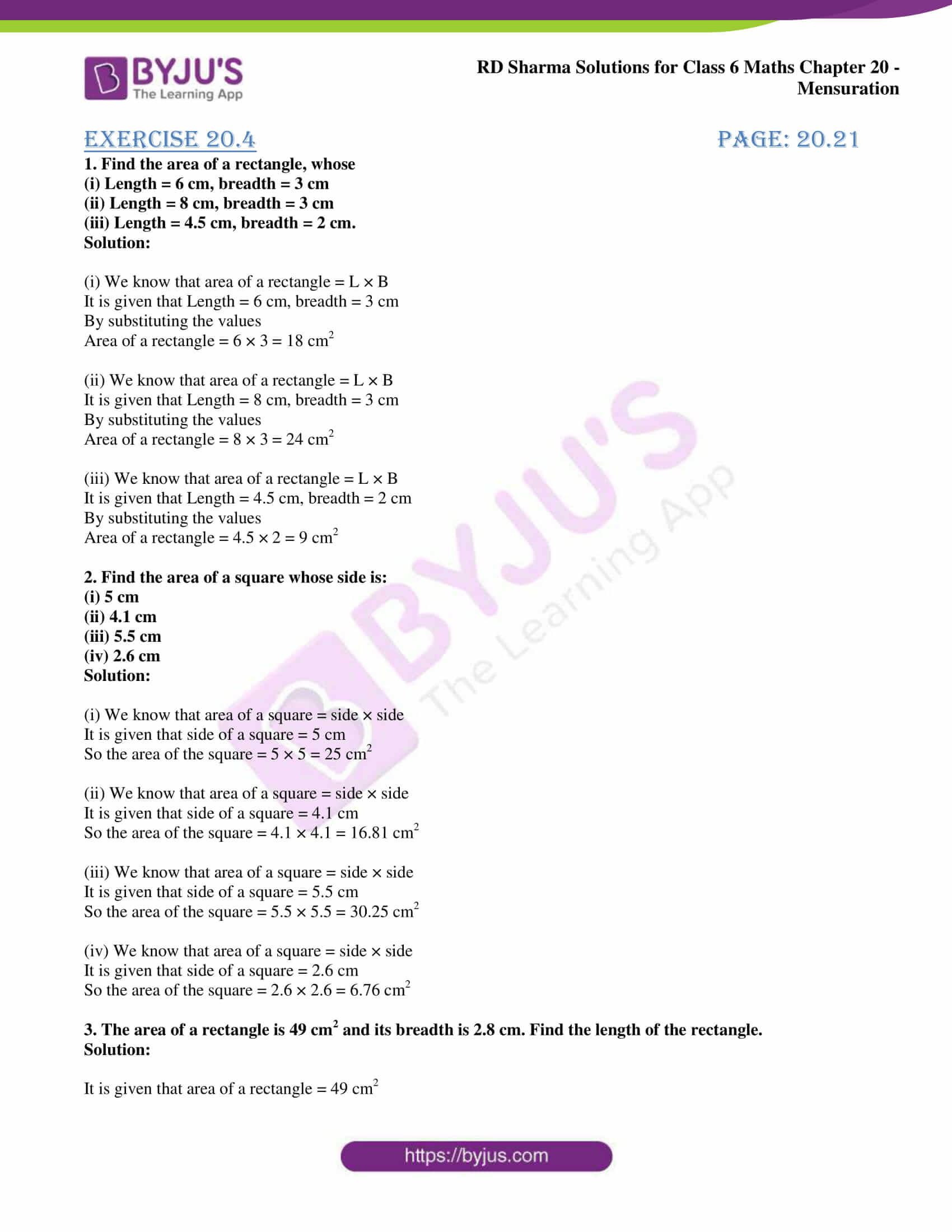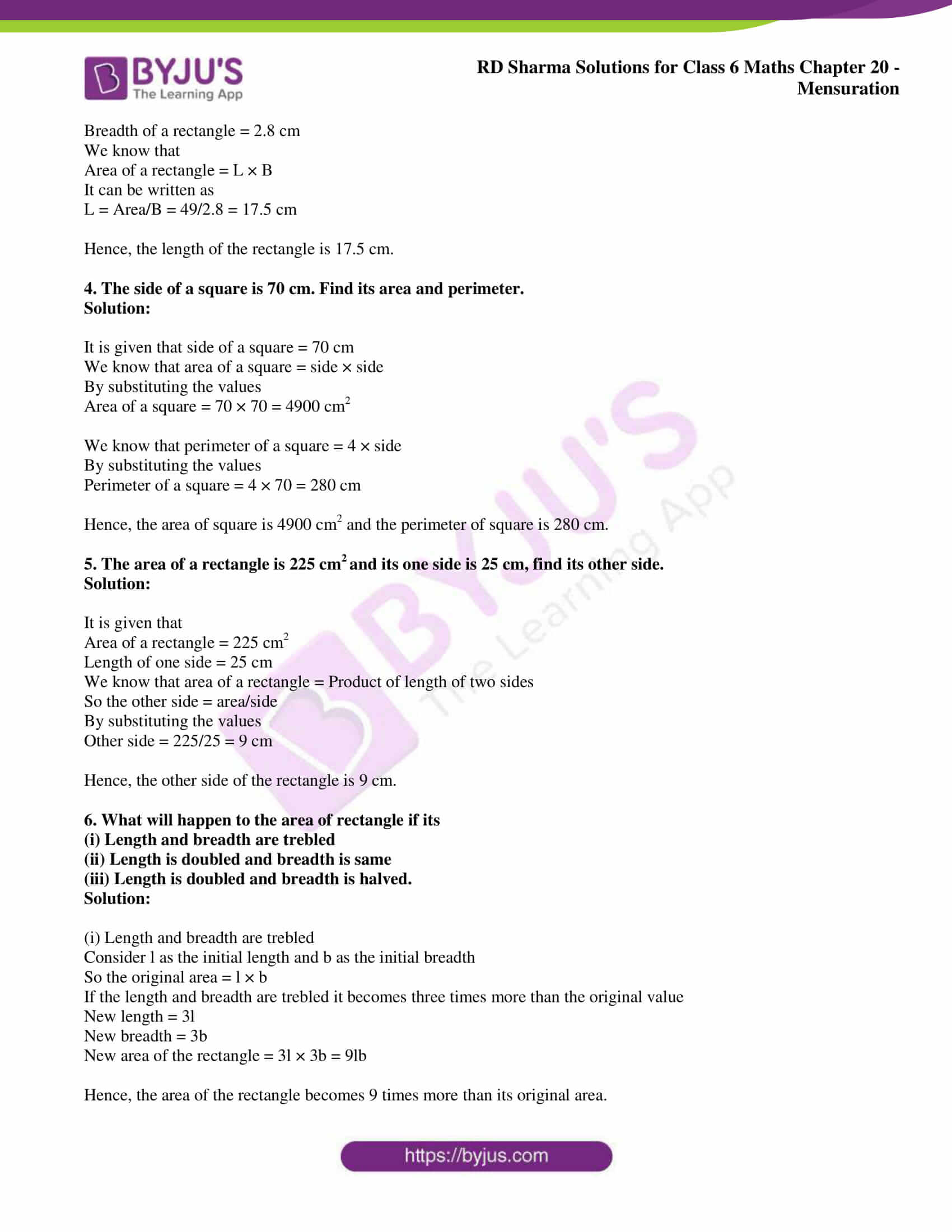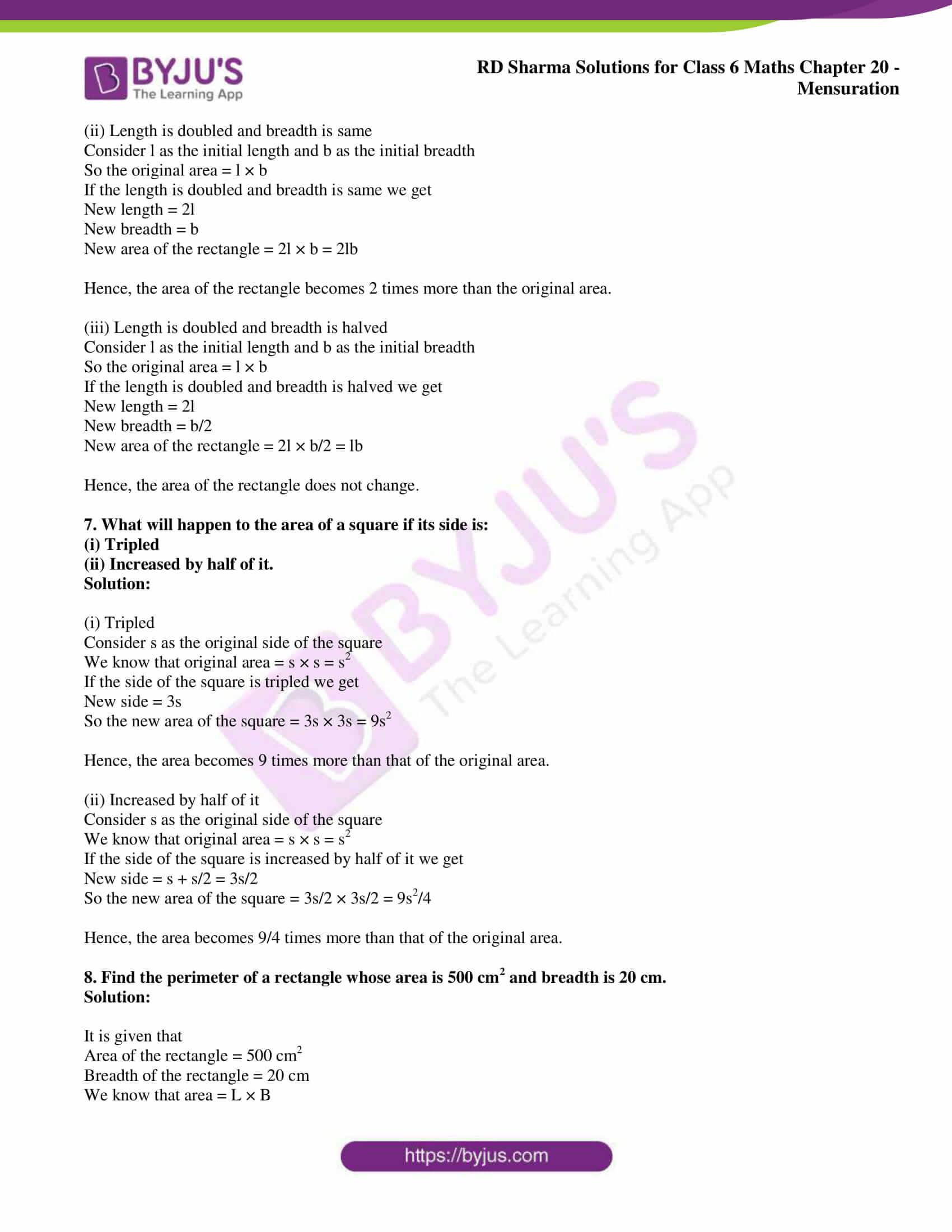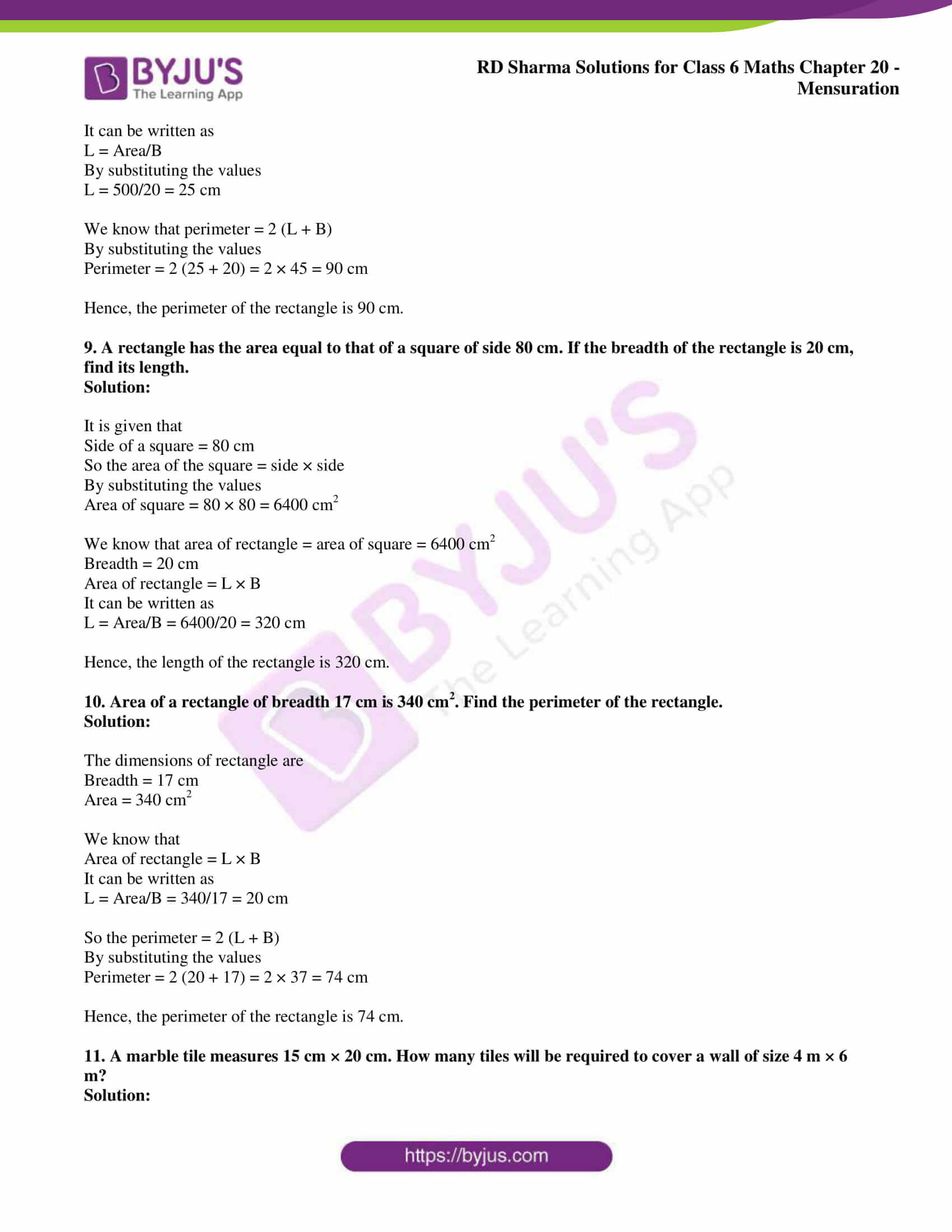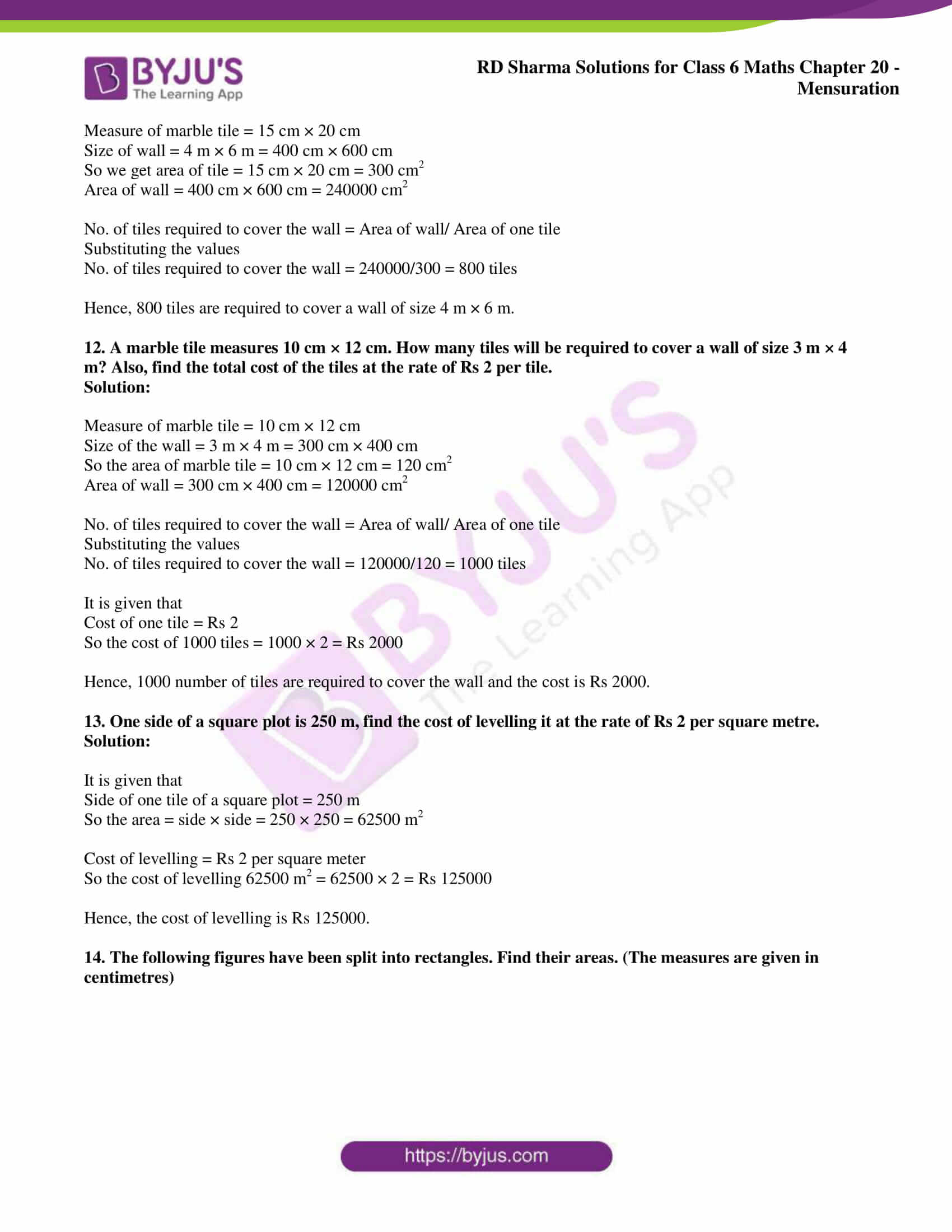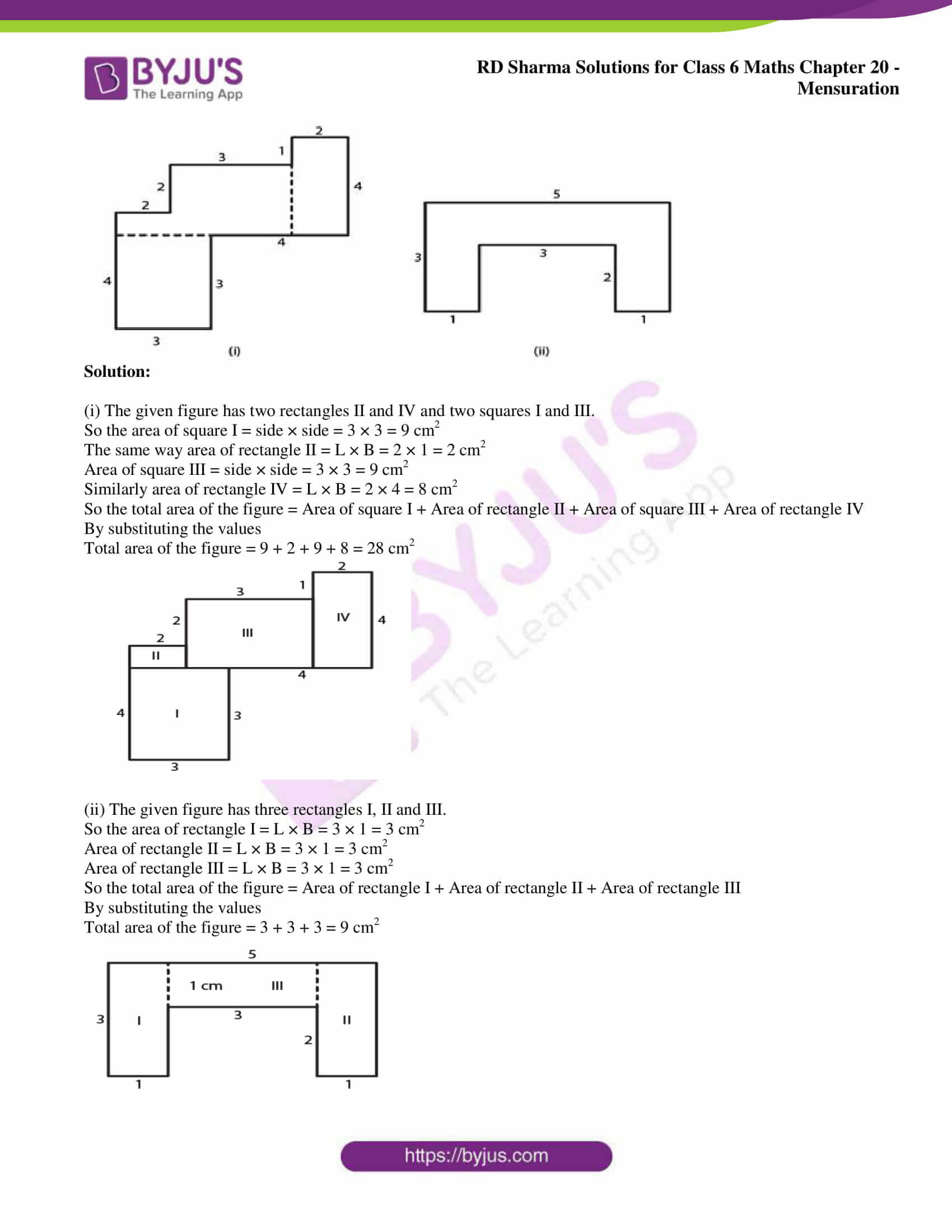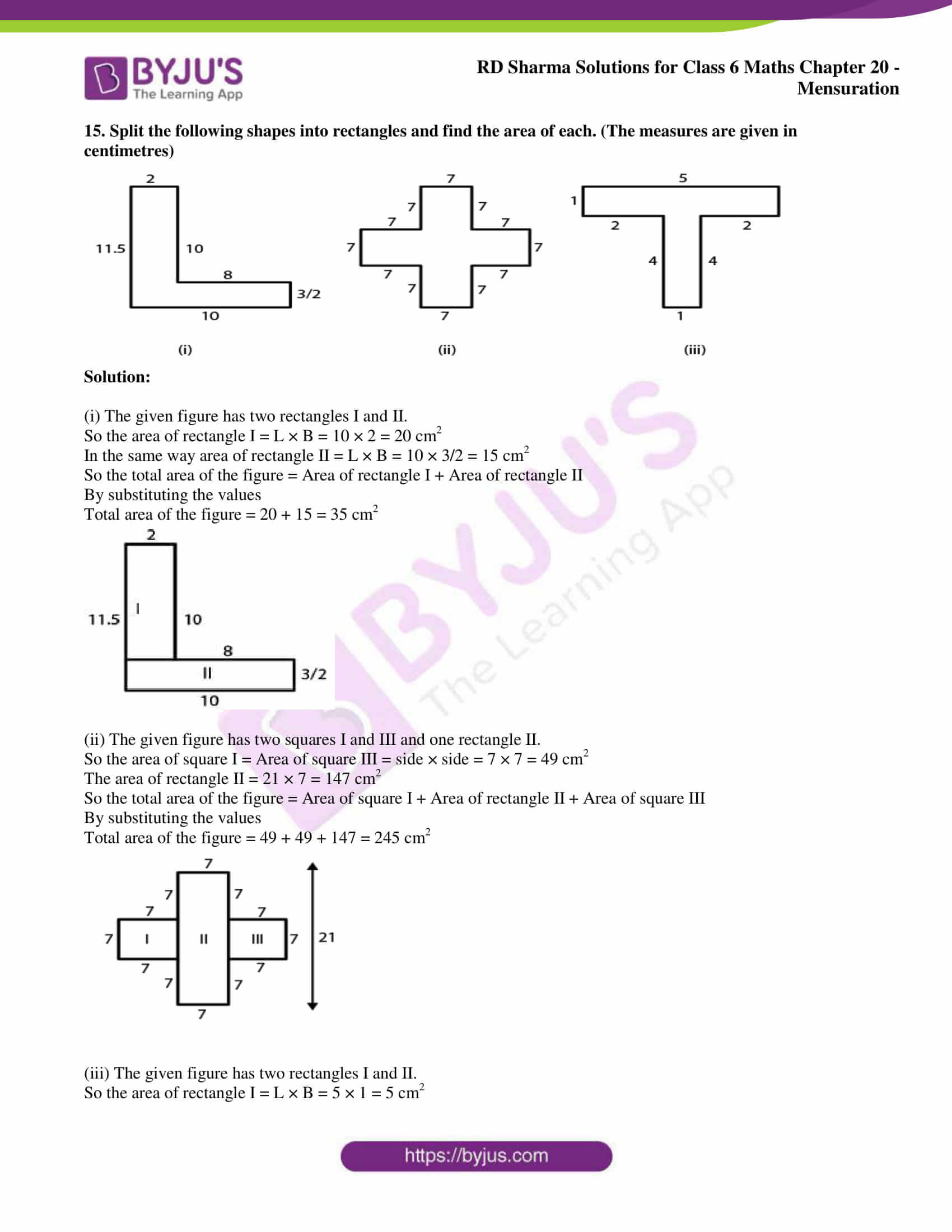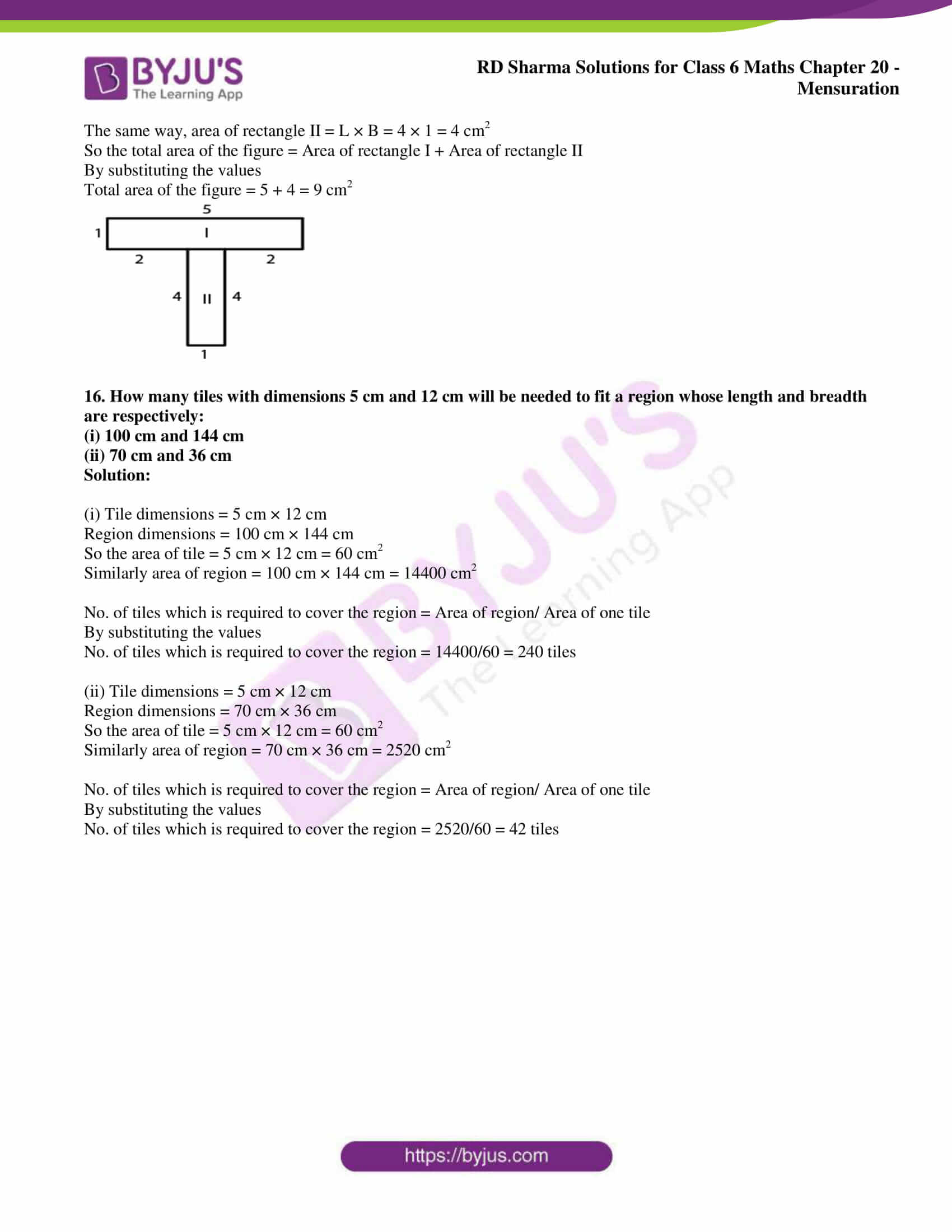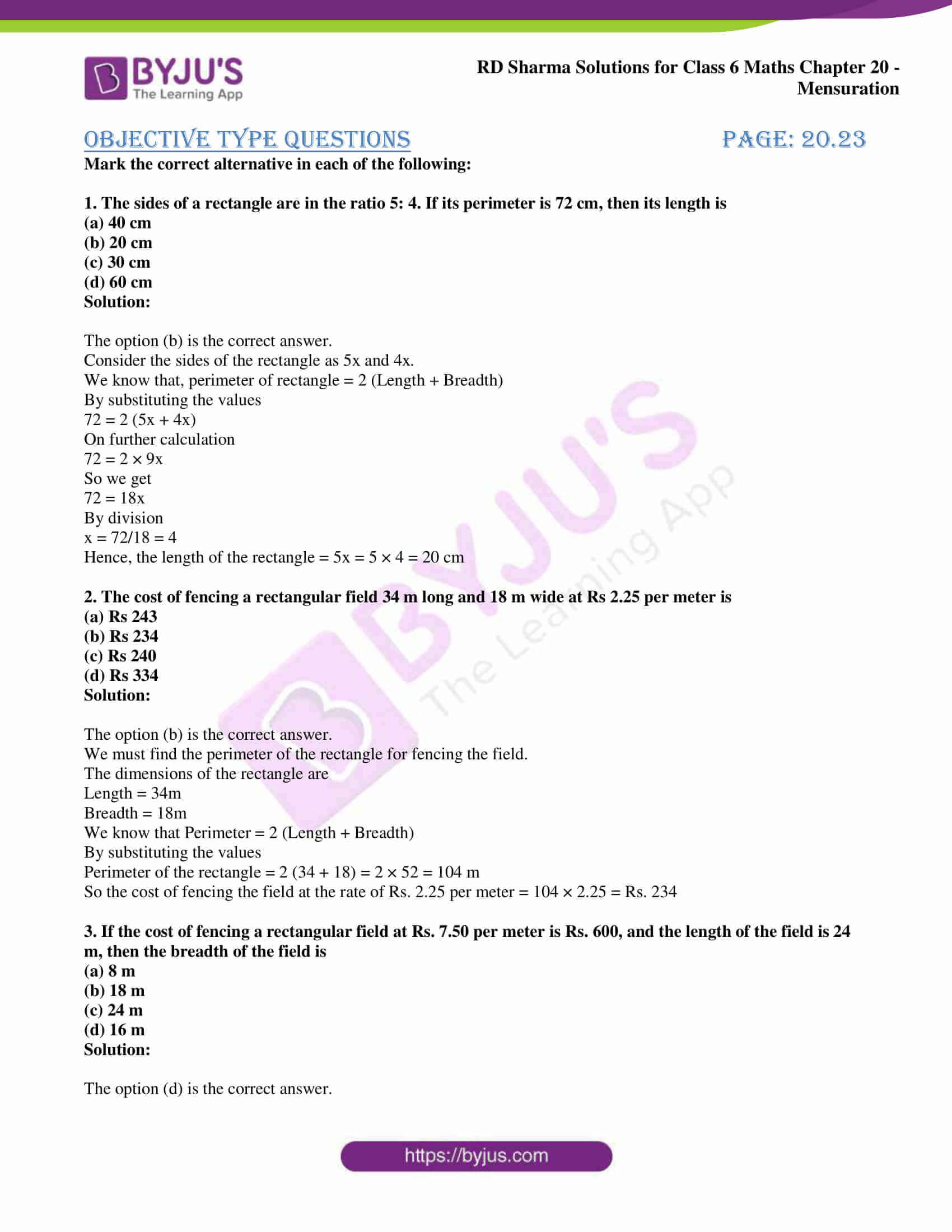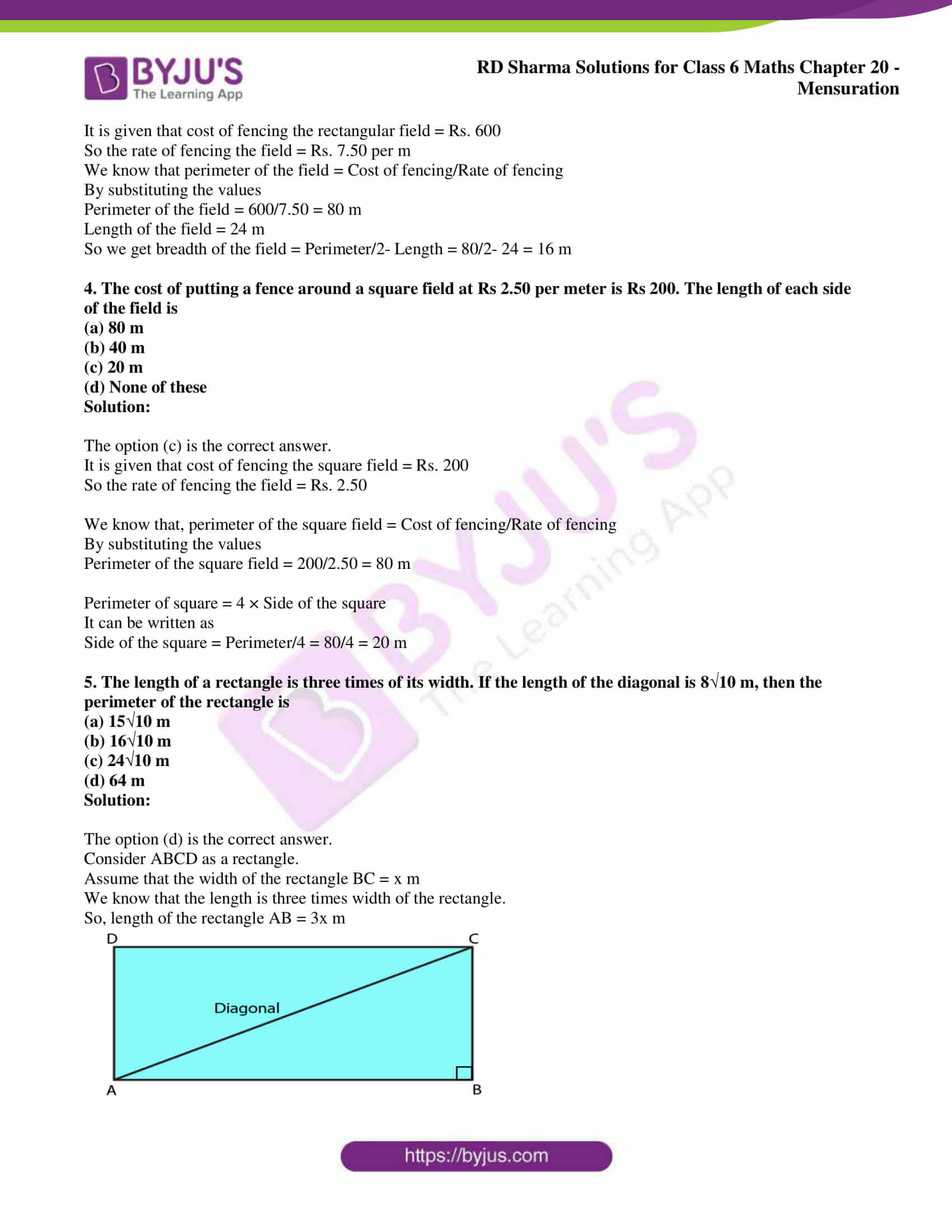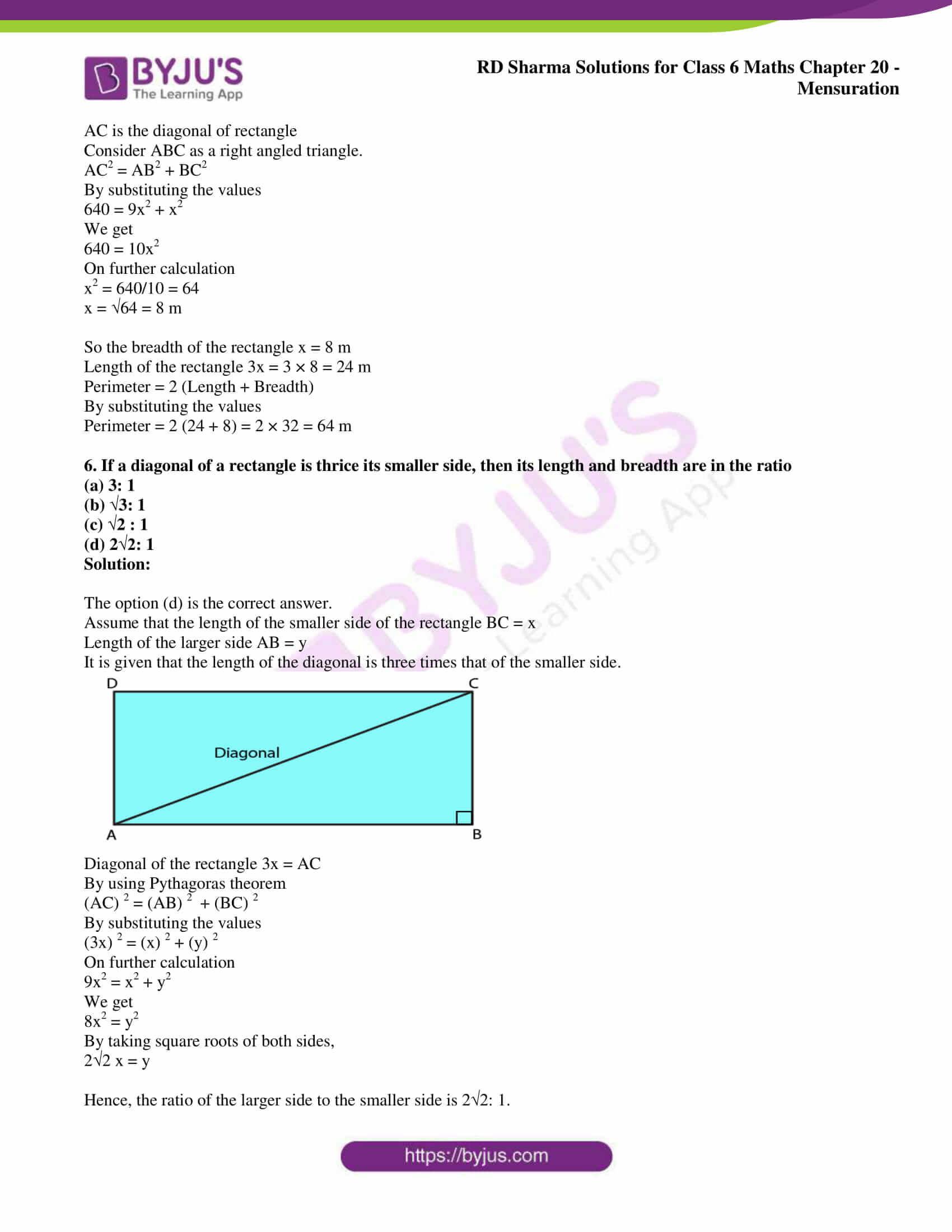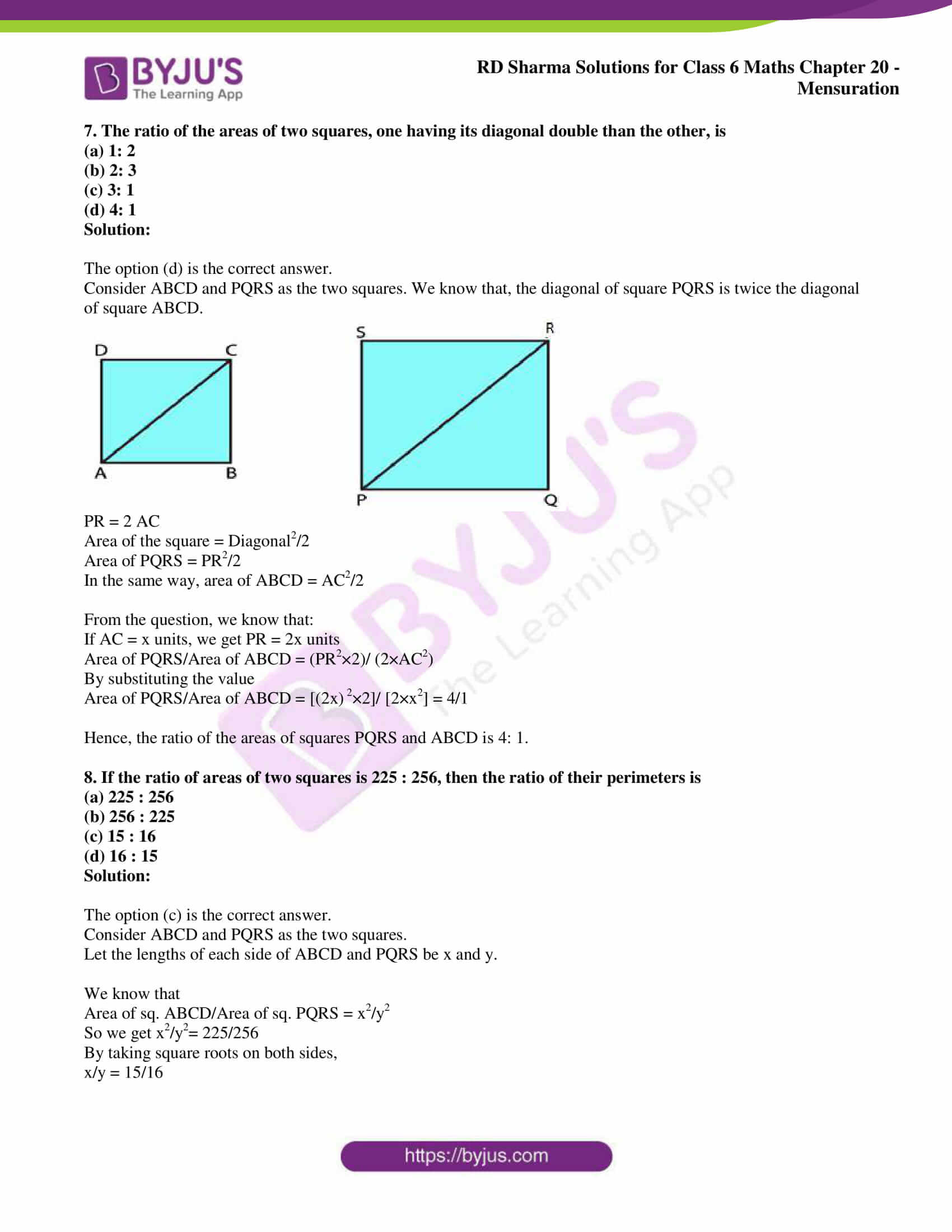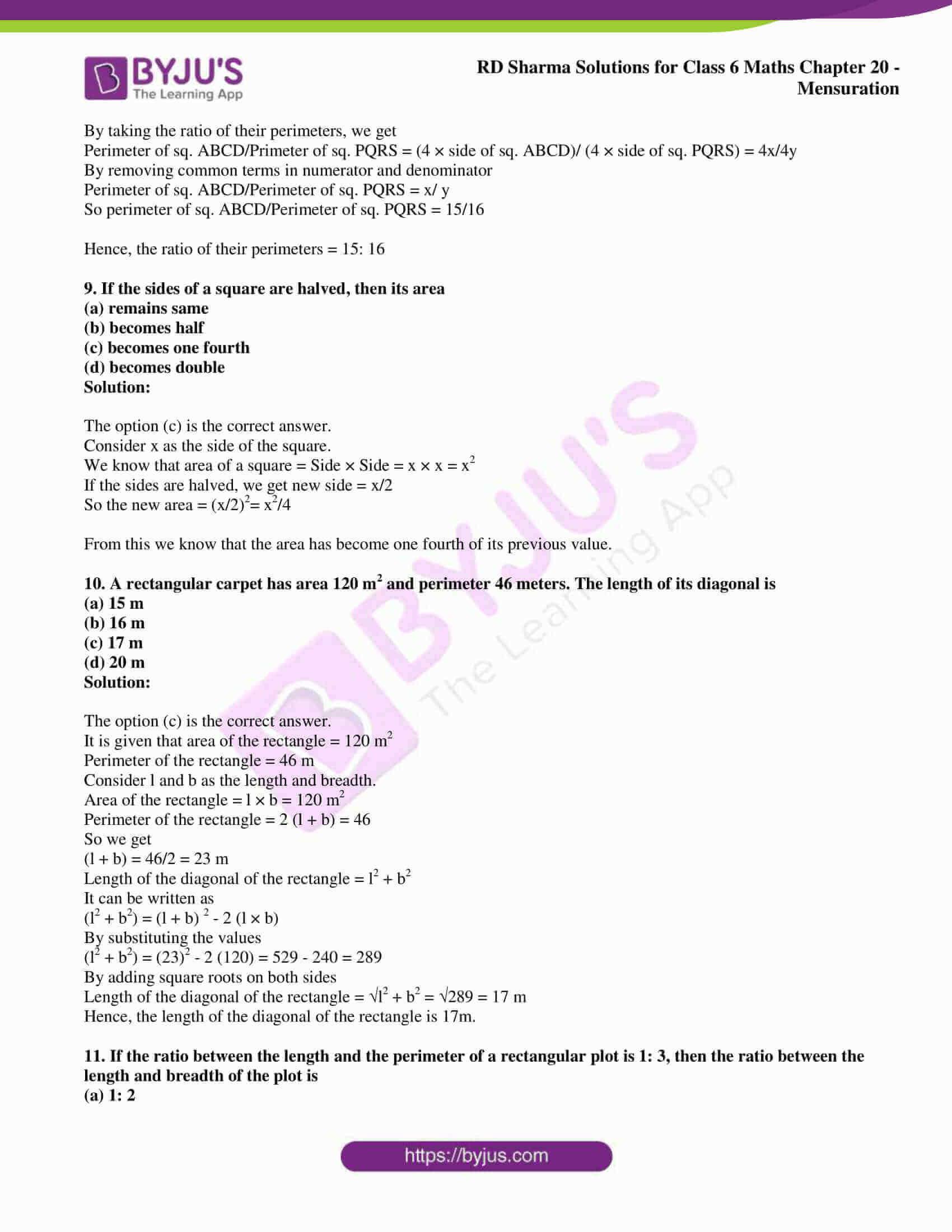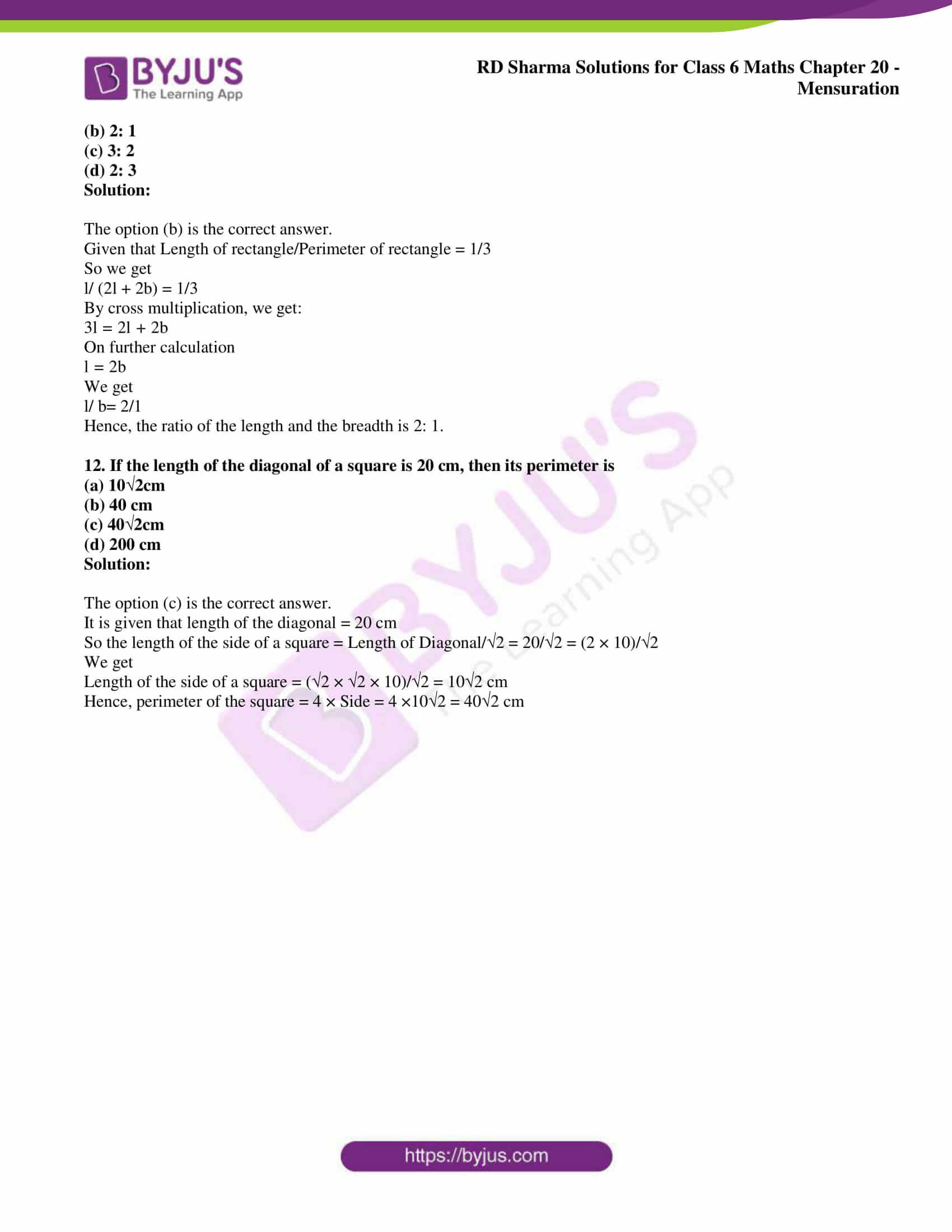## Access answers to Maths RD Sharma Solutions for Class 6 Chapter 20: Mensuration

### Exercise 20.1 page: 20.5

1. Which of the following are closed curves? Which of them are simple?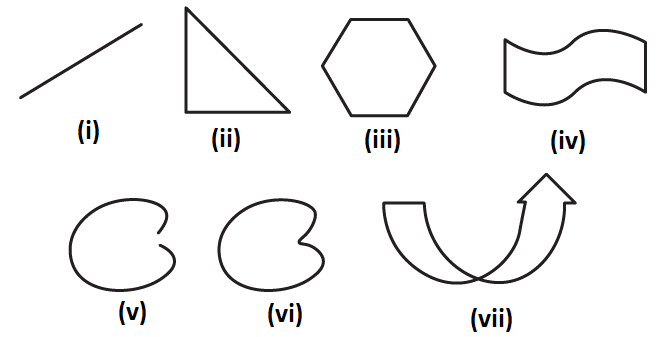Solution:

The figures (ii), (iii), (iv), (vi) and (vii) are closed curves and the figures (ii), (iii), (iv) and (vi) are simple closed curves.

2. Define perimeter of a closed figure.

Solution:

The length of the boundary of a closed figure is known as its perimeter.

3. Find the perimeter of each of the following shapes: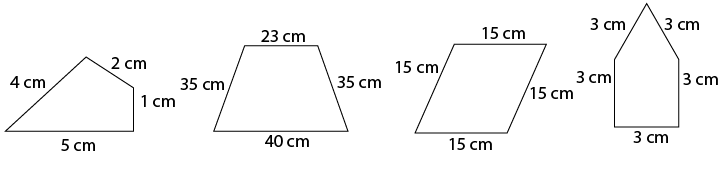Solution:

We know that perimeter is the sum of lengths of all the sides of a closed figure.

(i) Perimeter of the given figure = 4 + 2 + 1 + 5 = 12 cm

(ii) Perimeter of the given figure = 23 + 35 + 40 + 35 = 133 cm

(iii) Perimeter of the given figure = 15 + 15 + 15 + 15 = 60 cm

(iv) Perimeter of the given figure = 3 + 3 + 3 + 3 + 3 = 15 cm

### Exercise 20.2 page: 20.10

1. Find the perimeters of the rectangles whose lengths and breadths are given below:

(i) 7 cm, 5 cm

(ii) 5 cm, 4 cm

(iii) 7.5 cm, 4.5 cm

Solution:

(i) We know that the perimeter of a rectangle = 2 (L + B)

It is given that L = 7 cm and B = 5 cm

So the perimeter of a rectangle = 2 (7 + 5) = 2 × 12 = 24 cm

(ii) We know that the perimeter of a rectangle = 2 (L + B)

It is given that L = 5 cm and B = 4 cm

So the perimeter of a rectangle = 2 (5 + 4) = 2 × 9 = 18 cm

(iii) We know that the perimeter of a rectangle = 2 (L + B)

It is given that L = 7.5 cm and B = 4.5 cm

So the perimeter of a rectangle = 2 (7.5 + 4.5) = 2 × 12 = 24 cm

2. Find the perimeters of the squares whose sides are given below:

(i) 10 cm

(ii) 5 m

(iii) 115.5 cm

Solution:

(i) We know that the perimeter of a square = 4 × Length of one side

It is given that L = 10 cm

So the perimeter of a square = 4 × 10 = 40 cm

(ii) We know that the perimeter of a square = 4 × Length of one side

It is given that L = 5 m

So the perimeter of a square = 4 × 5 = 20 m

(iii) We know that the perimeter of a square = 4 × Length of one side

It is given that L = 115.5 cm

So the perimeter of a square = 4 × 115.5 = 462 cm

3. Find the side of the square whose perimeter is:

(i) 16 m

(ii) 40 cm

(iii) 22 cm

Solution:

(i) We know that side of a square = perimeter/ 4

It is given that perimeter = 16 m

So the side of the square = 16/4 = 4 m

(ii) We know that side of a square = perimeter/ 4

It is given that perimeter = 40 cm

So the side of the square = 40/4 = 10 cm

(iii) We know that side of a square = perimeter/ 4

It is given that perimeter = 22 cm

So the side of the square = 22/4 = 5.5 cm

4. Find the breadth of the rectangle whose perimeter is 360 cm and whose length is

(i) 116 cm

(ii) 140 cm

(iii) 102 cm

Solution:

We know that the perimeter of a rectangle = 2 (L + B)

So the breadth of the rectangle = perimeter/2 – length

(i) It is given that perimeter = 360 cm and length = 116 cm

So the breadth of the rectangle = 360/2 – 116 = 180 – 116 = 64 cm

(ii) It is given that perimeter = 360 cm and length = 140 cm

So the breadth of the rectangle = 360/2 – 140 = 180 – 140 = 40 cm

(iii) It is given that perimeter = 360 cm and length = 102 cm

So the breadth of the rectangle = 360/2 – 102 = 180 – 102 = 78 cm

5. A rectangular piece of lawn is 55 m wide and 98 m long. Find the length of the fence around it.

Solution:

The dimensions of lawn are

Length = 98 m

We know that

Perimeter of lawn = 2 (L + B)

By substituting the values

Perimeter of lawn = 2 (98 +55)

So we get

Perimeter of lawn = 2 × 153 = 306 m

Hence, the length of the fence around the lawn is 306 m.

6. The side of a square field is 65 m. What is the length of the fence required all around it?

Solution:

It is given that

Side of a square field = 65 m

So the perimeter of square field = 4 × side of the square

By substituting the values

Perimeter of square field = 4 × 65 = 260 m

Hence, the length of the fence required all around the square field is 260 m.

7. Two sides of a triangle are 15 cm and 20 cm. The perimeter of the triangle is 50 cm. What is the third side?

Solution:

It is given that

First side of triangle = 15 cm

Second side of triangle = 20 cm

In order to find the length of third side

We know that perimeter of a triangle is the sum of all three sides of a triangle

So the length of third side = perimeter of triangle – sum of length of other two sides

By substituting the values

Length of third side = 50 – (15 + 20) = 15 cm.

Hence, the length of third side is 15 cm.

8. A wire of length 20 m is to be folded in the form of a rectangle. How many rectangles can be formed by folding the wire if the sides are positive integers in metres?

Solution:

Given:

Length of wire 20 m is folded in the form of rectangle

So the perimeter = 20 m

It can be written as

2 (L + B) = 20 m

On further calculation

L + B = 10 m

If the sides are positive integers in metres the possible dimensions are (1m, 9m), (2m, 8m), (3m, 7m), (4m, 6m) and (5m, 5m)

Hence, five rectangles can be formed using the given wire.

9. A square piece of land has each side equal to 100 m. If 3 layers of metal wire has to be used to fence it, what is the length of the wire needed?

Solution:

It is given that

Each side of a square field = 100 m

We can find the wire required to fence the square field by determining the perimeter = 4 × each side of a square field

By substituting the values

Perimeter of the square field = 4 × 100 = 400 m

So the length of wire which is required to fence three layers is = 3 × 400 = 1200 m

Hence, the length of wire needed to fence 3 layers is 1200 m.

10. Shikha runs around a square of side 75 m. Priya runs around a rectangle with length 60 m and breadth 45 m. Who covers the smaller distance?

Solution:

It is given that

Shikha runs around a square of side = 75 m

So the perimeter = 4 × 75 = 300 m

Priya runs around a rectangle having

Length = 60 m

So the distance covered can be found from the perimeter = 2 (L + B)

By substituting the values

Perimeter = 2 (60 + 45) = 2 × 105 = 210 m

Hence, Priya covers the smaller distance of 210 m.

11. The dimensions of a photographs are 30 cm × 20 cm. What length of wooden frame is needed to frame the picture?

Solution:

It is given that

Dimensions of a photographs = 30 cm × 20 cm

So the required length of the wooden frame can be determined from the perimeter of the photograph = 2 (L + B)

By substituting the values = 2 (30 + 20) = 2 × 50 = 100 cm

Hence, the length of the wooden frame required to frame the picture is 100 cm.

12. The length of a rectangular field is 100 m. If the perimeter is 300 m, what is its breadth?

Solution:

The dimensions of rectangular field are

Length = 100 m

Perimeter = 300 m

We know that perimeter = 2 (L + B)

It can be written as

By substituting the values

Breadth = (300-200)/2 = 100/2 = 50 m

Hence, the breadth of the rectangular field is 50 m.

13. To fix fence wires in a garden, 70 m long and 50 m wide, Arvind bought metal pipes for posts. He fixed a post every 5 metres apart. Each post was 2 m long. What is the total length of the pipes he bought for the posts?

Solution:

The dimensions of garden are

Length = 70 m

So the perimeter = 2 (L + B)

By substituting the values

Perimeter = 2 (70 + 50) = 2 × 120 = 240 m

Given:

Arvind fixes a post every 5 metres apart

No. of posts required = 240/5 = 48

The length of each post = 2 m

So the total length of the pipe required = 48 × 2 = 96 m

Hence, the total length of the pipes he bought for the posts is 96 m.

14. Find the cost of fencing a rectangular park of length 175 m and breadth 125 m at the rate of Rs 12 per meter.

Solution:

The dimensions of the rectangular park are

Length = 175 m

So the perimeter = 2 (L + B)

By substituting the values

Perimeter = 2 (175 + 125) = 2 × 300 = 600 m

It is given that the cost of fencing = Rs 12 per meter

So the total cost of fencing = 12 × 600 = Rs 7200

Hence, the cost of fencing a rectangular park is Rs 7200.

15. The perimeter of a regular pentagon is 100 cm. How long is each side?

Solution:

We know that a regular pentagon is a closed polygon having 5 sides of same length.

It is given that

Perimeter of a regular pentagon = 100 cm

It can be written as

Perimeter = 5 × side of the regular pentagon

So we get

Side of the regular pentagon = Perimeter/5

By substituting the values

Side of the regular pentagon = 100/5 = 20 cm

Hence, the side of the regular pentagon measures 20 cm.

16. Find the perimeter of a regular hexagon with each side measuring 8 m.

Solution:

We know that a regular hexagon is a closed polygon which has six sides of same length.

It is given that

Side of the regular hexagon = 8 m

So we get

Perimeter = 6 × side of the regular hexagon

By substituting the values

Perimeter = 6 × 8 = 48 m

Hence, the perimeter of a regular hexagon is 48 m.

17. A rectangular piece of land measure 0.7 km by 0.5 km. Each side is to be fenced with four rows of wires. What length of the wire is needed?

Solution:

It is given that

Measure of rectangular piece of land = 0.7 km × 0.5 km

We know that

Perimeter = 2 (L + B)

By substituting the values

Perimeter = 2 (0.7 + 0.5) = 2 × 1.2 = 2.4 km

The above obtained perimeter = one row of wire needed to fence the rectangular piece of land

So the length of wire needed to fence the land with 4 rows of wire = 4 × 2.4 = 9.6 km

Hence, the length of wire needed is 9.6 km.

18. Avneet buys 9 square paving slabs, each with a side of ½ m. He lays them in the form of a square.

(i) What is the perimeter of his arrangement?

(ii) Shari does not like his arrangement. She gets him to lay them out like a cross. What is the perimeter of her arrangement?

(iii) Which has greater perimeter?

(iv) Avneet wonders, if there is a way of getting an even greater perimeter. Can you find a way of doing this? (The paving slabs must meet along complete edges they cannot be broken)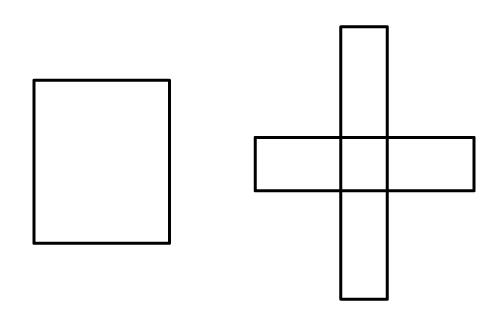Solution:

(i) It is given that length of each side of the slab = ½ m

One side of the square is formed by three slabs in a square arrangement

Length of side = 3 × ½ = 3/2 m

So the perimeter of the square arrangement = 4 × 3/2 = 6 m

(ii) From the figure, cross arrangement has 8 sides which form periphery of the arrangement and measure 1 m each.

It also has 4 sides which measure ½ m each

Perimeter of the cross arrangement = 1 + ½ + 1 + 1 + ½ + 1 + 1 + ½ + 1 + 1 + ½ + 1 = 8 + 2 = 10 m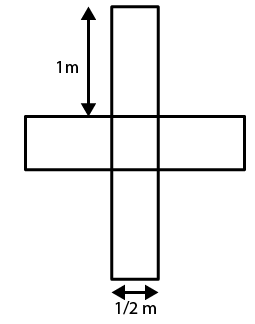(iii) We know that

Perimeter of cross arrangement = 10 m

Perimeter of square arrangement = 6 m

Hence, the perimeter of cross arrangement is greater than the perimeter of square arrangement.

(iv) No, Avneet cannot arrange the slabs having perimeter more than 10 m.

### Exercise 20.3 page: 20.14

1. The following figures are drawn on a squared paper. Count the number of squares enclosed by each figure and find its area, taking the area of each square as 1 cm2. (Fig. 20.25).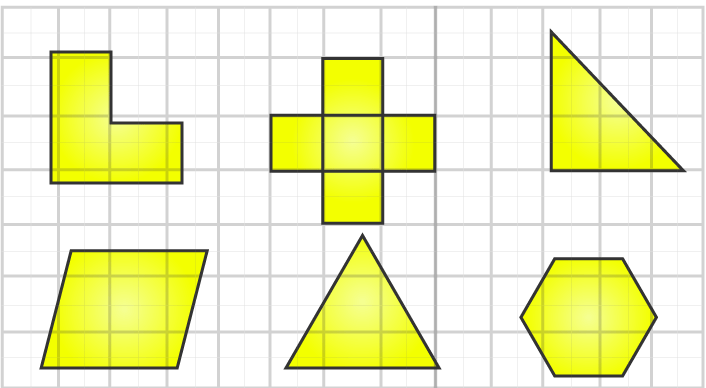Solution:

(i) The given shape has 16 complete squares.

It is given that area of one square = 1 cm2

So the area of the given shape = 16 × 1 = 16 cm2

(ii) The given shape has 36 complete squares.

It is given that area of one square = 1 cm2

So the area of the given shape = 36 × 1 = 36 cm2

(iii) The given shape has 15 complete and 6 half squares.

It is given that area of one square = 1 cm2

So the area of the given shape = 15 + 6 × 12 = 18 cm2

(iv) The given shape has 20 complete and 8 half squares.

It is given that area of one square = 1 cm2

So the area of the given shape = 20 + 8 × 12 = 24 cm2

(v) The given shape has 13 complete, 8 more than half and 7 less than half squares.

It is given that area of one square = 1 cm2

So the area of the given shape = 13 + 8 × 1 = 21 cm2

(vi) The given shape has 8 complete, 6 more than half and 4 less than half squares.

It is given that area of one square = 1 cm2

So the area of the given shape = 8 + 6 × 1 = 14 cm2

2. On a squared paper, draw (i) a rectangle, (ii) a triangle (iii) any irregular closed figure. Find the approximate area of each by counting the number of squares complete, more than half and exactly half.

Solution:

(i) A rectangle

The given shape has 18 complete squares

Assume that area of one square = 1 cm2

So the area of the rectangle = 18 × 1 = 18 cm2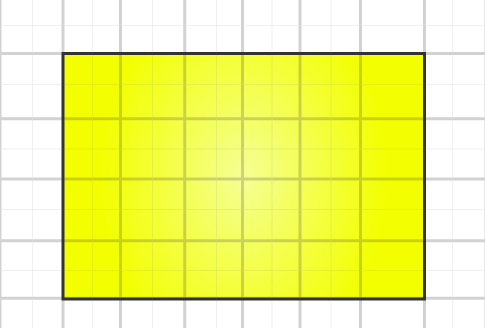(ii) A triangle

The given shape has 4 complete, 6 more than half and 6 less than half squares.

Assume that area of one square = 1 cm2

So the area of the square = 4 + 6 × 1 = 10 cm2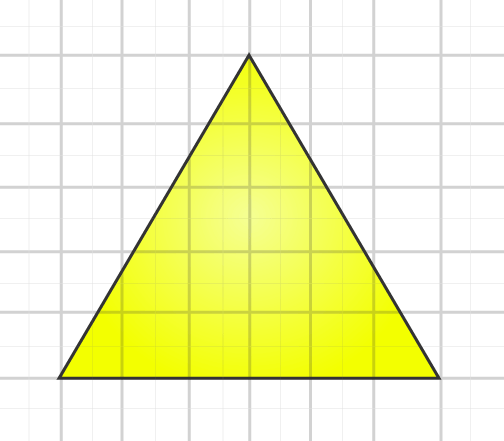(iii) Any irregular figure

The given shape has 10 complete, 1 exactly half, 7 more than half and 6 less than half squares.

Assume that area of one square = 1 cm2

So the area of the shape = 10 + 1 × 12 + 7 × 1 = 17.5 cm2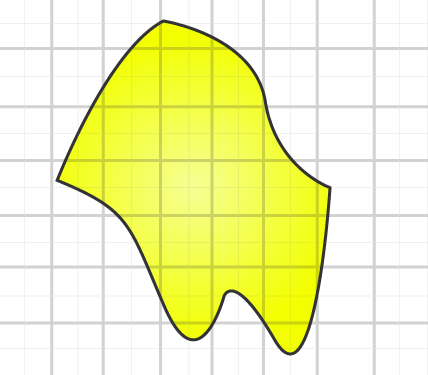3. Draw any circle on the graph paper. Count the squares and use them to estimate the area of the circular region.

Solution: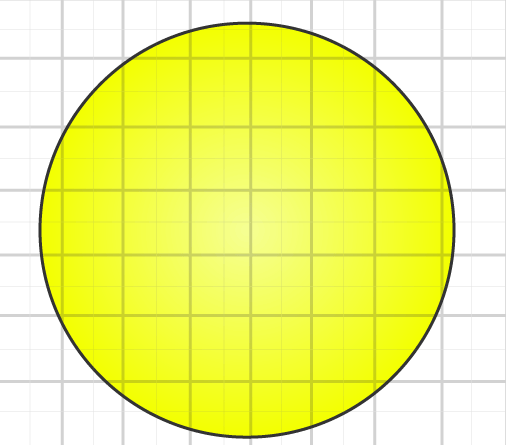The given circles has 21 complete, 15 more than half and 8 less than half squares.

Assume that area of one square = 1 cm2

By neglecting less than half squares, we get

Area of the circle = 21 + 15 = 36 cm2

4. Use tracing paper and centimetre graph paper to compare the areas of the following pairs of figures: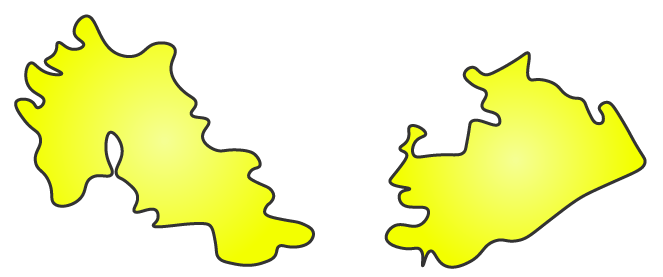Solution: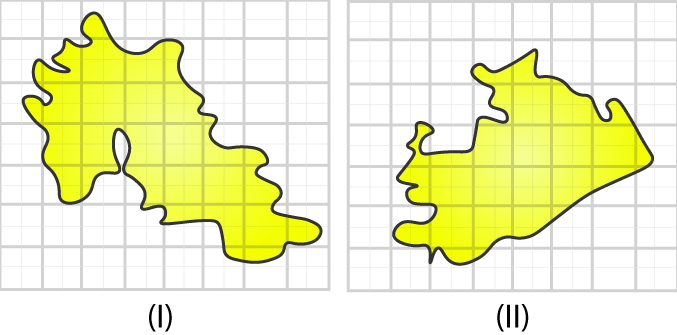With the help of tracing paper trace both the figures on a graph

Figure (i) has 4 complete, 9 more than half and 9 less than half squares.

Assume that area of one square = 1 cm2

By neglecting less than half squares, we get

Area of the shape = 4 + 9 = 13 cm2

Figure (ii) has 8 complete, 11 more than half and 10 less than half squares.

Assume that area of one square = 1 cm2

By neglecting less than half squares, we get

Area of the shape = 8 + 11 = 19 cm2

By comparing the areas of both the shapes, we know that the figure (ii) has area greater than that of figure (i).

### Exercise 20.4 page: 20.21

1. Find the area of a rectangle, whose

(i) Length = 6 cm, breadth = 3 cm

(ii) Length = 8 cm, breadth = 3 cm

(iii) Length = 4.5 cm, breadth = 2 cm.

Solution:

(i) We know that area of a rectangle = L × B

It is given that Length = 6 cm, breadth = 3 cm

By substituting the values

Area of a rectangle = 6 × 3 = 18 cm2

(ii) We know that area of a rectangle = L × B

It is given that Length = 8 cm, breadth = 3 cm

By substituting the values

Area of a rectangle = 8 × 3 = 24 cm2

(iii) We know that area of a rectangle = L × B

It is given that Length = 4.5 cm, breadth = 2 cm

By substituting the values

Area of a rectangle = 4.5 × 2 = 9 cm2

2. Find the area of a square whose side is:

(i) 5 cm

(ii) 4.1 cm

(iii) 5.5 cm

(iv) 2.6 cm

Solution:

(i) We know that area of a square = side × side

It is given that side of a square = 5 cm

So the area of the square = 5 × 5 = 25 cm2

(ii) We know that area of a square = side × side

It is given that side of a square = 4.1 cm

So the area of the square = 4.1 × 4.1 = 16.81 cm2

(iii) We know that area of a square = side × side

It is given that side of a square = 5.5 cm

So the area of the square = 5.5 × 5.5 = 30.25 cm2

(iv) We know that area of a square = side × side

It is given that side of a square = 2.6 cm

So the area of the square = 2.6 × 2.6 = 6.76 cm2

3. The area of a rectangle is 49 cm2 and its breadth is 2.8 cm. Find the length of the rectangle.

Solution:

It is given that area of a rectangle = 49 cm2

Breadth of a rectangle = 2.8 cm

We know that

Area of a rectangle = L × B

It can be written as

L = Area/B = 49/2.8 = 17.5 cm

Hence, the length of the rectangle is 17.5 cm.

4. The side of a square is 70 cm. Find its area and perimeter.

Solution:

It is given that side of a square = 70 cm

We know that area of a square = side × side

By substituting the values

Area of a square = 70 × 70 = 4900 cm2

We know that perimeter of a square = 4 × side

By substituting the values

Perimeter of a square = 4 × 70 = 280 cm

Hence, the area of square is 4900 cm2 and the perimeter of square is 280 cm.

5. The area of a rectangle is 225 cm2 and its one side is 25 cm, find its other side.

Solution:

It is given that

Area of a rectangle = 225 cm2

Length of one side = 25 cm

We know that area of a rectangle = Product of length of two sides

So the other side = area/side

By substituting the values

Other side = 225/25 = 9 cm

Hence, the other side of the rectangle is 9 cm.

6. What will happen to the area of rectangle if its

(i) Length and breadth are trebled

(ii) Length is doubled and breadth is same

(iii) Length is doubled and breadth is halved.

Solution:

(i) Length and breadth are trebled

Consider l as the initial length and b as the initial breadth

So the original area = l × b

If the length and breadth are trebled it becomes three times more than the original value

New length = 3l

New area of the rectangle = 3l × 3b = 9lb

Hence, the area of the rectangle becomes 9 times more than its original area.

(ii) Length is doubled and breadth is same

Consider l as the initial length and b as the initial breadth

So the original area = l × b

If the length is doubled and breadth is same we get

New length = 2l

New area of the rectangle = 2l × b = 2lb

Hence, the area of the rectangle becomes 2 times more than the original area.

(iii) Length is doubled and breadth is halved

Consider l as the initial length and b as the initial breadth

So the original area = l × b

If the length is doubled and breadth is halved we get

New length = 2l

New area of the rectangle = 2l × b/2 = lb

Hence, the area of the rectangle does not change.

7. What will happen to the area of a square if its side is:

(i) Tripled

(ii) Increased by half of it.

Solution:

(i) Tripled

Consider s as the original side of the square

We know that original area = s × s = s2

If the side of the square is tripled we get

New side = 3s

So the new area of the square = 3s × 3s = 9s2

Hence, the area becomes 9 times more than that of the original area.

(ii) Increased by half of it

Consider s as the original side of the square

We know that original area = s × s = s2

If the side of the square is increased by half of it we get

New side = s + s/2 = 3s/2

So the new area of the square = 3s/2 × 3s/2 = 9s2/4

Hence, the area becomes 9/4 times more than that of the original area.

8. Find the perimeter of a rectangle whose area is 500 cm2 and breadth is 20 cm.

Solution:

It is given that

Area of the rectangle = 500 cm2

Breadth of the rectangle = 20 cm

We know that area = L × B

It can be written as

L = Area/B

By substituting the values

L = 500/20 = 25 cm

We know that perimeter = 2 (L + B)

By substituting the values

Perimeter = 2 (25 + 20) = 2 × 45 = 90 cm

Hence, the perimeter of the rectangle is 90 cm.

9. A rectangle has the area equal to that of a square of side 80 cm. If the breadth of the rectangle is 20 cm, find its length.

Solution:

It is given that

Side of a square = 80 cm

So the area of the square = side × side

By substituting the values

Area of square = 80 × 80 = 6400 cm2

We know that area of rectangle = area of square = 6400 cm2

Area of rectangle = L × B

It can be written as

L = Area/B = 6400/20 = 320 cm

Hence, the length of the rectangle is 320 cm.

10. Area of a rectangle of breadth 17 cm is 340 cm2. Find the perimeter of the rectangle.

Solution:

The dimensions of rectangle are

Area = 340 cm2

We know that

Area of rectangle = L × B

It can be written as

L = Area/B = 340/17 = 20 cm

So the perimeter = 2 (L + B)

By substituting the values

Perimeter = 2 (20 + 17) = 2 × 37 = 74 cm

Hence, the perimeter of the rectangle is 74 cm.

11. A marble tile measures 15 cm × 20 cm. How many tiles will be required to cover a wall of size 4 m × 6 m?

Solution:

Measure of marble tile = 15 cm × 20 cm

Size of wall = 4 m × 6 m = 400 cm × 600 cm

So we get area of tile = 15 cm × 20 cm = 300 cm2

Area of wall = 400 cm × 600 cm = 240000 cm2

No. of tiles required to cover the wall = Area of wall/ Area of one tile

Substituting the values

No. of tiles required to cover the wall = 240000/300 = 800 tiles

Hence, 800 tiles are required to cover a wall of size 4 m × 6 m.

12. A marble tile measures 10 cm × 12 cm. How many tiles will be required to cover a wall of size 3 m × 4 m? Also, find the total cost of the tiles at the rate of Rs 2 per tile.

Solution:

Measure of marble tile = 10 cm × 12 cm

Size of the wall = 3 m × 4 m = 300 cm × 400 cm

So the area of marble tile = 10 cm × 12 cm = 120 cm2

Area of wall = 300 cm × 400 cm = 120000 cm2

No. of tiles required to cover the wall = Area of wall/ Area of one tile

Substituting the values

No. of tiles required to cover the wall = 120000/120 = 1000 tiles

It is given that

Cost of one tile = Rs 2

So the cost of 1000 tiles = 1000 × 2 = Rs 2000

Hence, 1000 number of tiles are required to cover the wall and the cost is Rs 2000.

13. One side of a square plot is 250 m, find the cost of levelling it at the rate of Rs 2 per square metre.

Solution:

It is given that

Side of one tile of a square plot = 250 m

So the area = side × side = 250 × 250 = 62500 m2

Cost of levelling = Rs 2 per square meter

So the cost of levelling 62500 m2 = 62500 × 2 = Rs 125000

Hence, the cost of levelling is Rs 125000.

14. The following figures have been split into rectangles. Find their areas. (The measures are given in centimetres)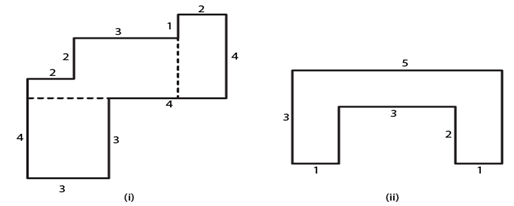Solution:

(i) The given figure has two rectangles II and IV and two squares I and III.

So the area of square I = side × side = 3 × 3 = 9 cm2

The same way area of rectangle II = L × B = 2 × 1 = 2 cm2

Area of square III = side × side = 3 × 3 = 9 cm2

Similarly area of rectangle IV = L × B = 2 × 4 = 8 cm2

So the total area of the figure = Area of square I + Area of rectangle II + Area of square III + Area of rectangle IV

By substituting the values

Total area of the figure = 9 + 2 + 9 + 8 = 28 cm2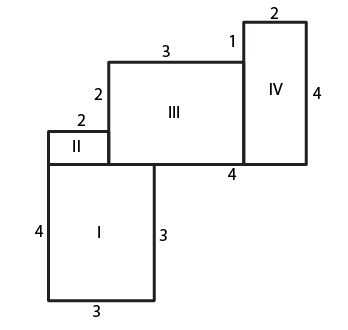(ii) The given figure has three rectangles I, II and III.

So the area of rectangle I = L × B = 3 × 1 = 3 cm2

Area of rectangle II = L × B = 3 × 1 = 3 cm2

Area of rectangle III = L × B = 3 × 1 = 3 cm2

So the total area of the figure = Area of rectangle I + Area of rectangle II + Area of rectangle III

By substituting the values

Total area of the figure = 3 + 3 + 3 = 9 cm2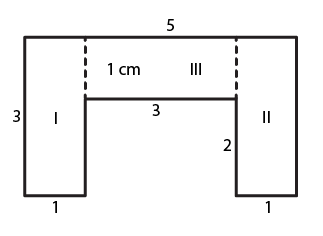15. Split the following shapes into rectangles and find the area of each. (The measures are given in centimetres)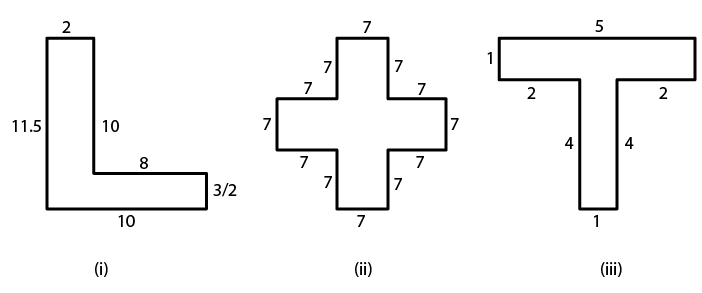Solution:

(i) The given figure has two rectangles I and II.

So the area of rectangle I = L × B = 10 × 2 = 20 cm2

In the same way area of rectangle II = L × B = 10 × 3/2 = 15 cm2

So the total area of the figure = Area of rectangle I + Area of rectangle II

By substituting the values

Total area of the figure = 20 + 15 = 35 cm2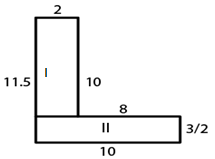(ii) The given figure has two squares I and III and one rectangle II.

So the area of square I = Area of square III = side × side = 7 × 7 = 49 cm2

The area of rectangle II = 21 × 7 = 147 cm2

So the total area of the figure = Area of square I + Area of rectangle II + Area of square III

By substituting the values

Total area of the figure = 49 + 49 + 147 = 245 cm2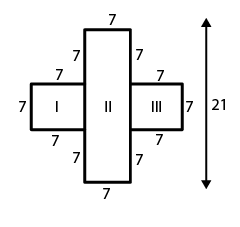(iii) The given figure has two rectangles I and II.

So the area of rectangle I = L × B = 5 × 1 = 5 cm2

The same way, area of rectangle II = L × B = 4 × 1 = 4 cm2

So the total area of the figure = Area of rectangle I + Area of rectangle II

By substituting the values

Total area of the figure = 5 + 4 = 9 cm2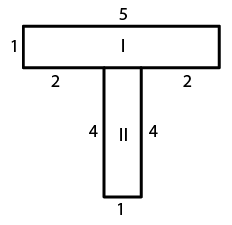16. How many tiles with dimensions 5 cm and 12 cm will be needed to fit a region whose length and breadth are respectively:

(i) 100 cm and 144 cm

(ii) 70 cm and 36 cm

Solution:

(i) Tile dimensions = 5 cm × 12 cm

Region dimensions = 100 cm × 144 cm

So the area of tile = 5 cm × 12 cm = 60 cm2

Similarly area of region = 100 cm × 144 cm = 14400 cm2

No. of tiles which is required to cover the region = Area of region/ Area of one tile

By substituting the values

No. of tiles which is required to cover the region = 14400/60 = 240 tiles

(ii) Tile dimensions = 5 cm × 12 cm

Region dimensions = 70 cm × 36 cm

So the area of tile = 5 cm × 12 cm = 60 cm2

Similarly area of region = 70 cm × 36 cm = 2520 cm2

No. of tiles which is required to cover the region = Area of region/ Area of one tile

By substituting the values

No. of tiles which is required to cover the region = 2520/60 = 42 tiles

### Objective Type Questions page: 20.23

Mark the correct alternative in each of the following:

1. The sides of a rectangle are in the ratio 5: 4. If its perimeter is 72 cm, then its length is
(a) 40 cm
(b) 20 cm
(c) 30 cm
(d) 60 cm

Solution:

The option (b) is the correct answer.

Consider the sides of the rectangle as 5x and 4x.

We know that, perimeter of rectangle = 2 (Length + Breadth)

By substituting the values

72 = 2 (5x + 4x)

On further calculation

72 = 2 × 9x

So we get

72 = 18x

By division

x = 72/18 = 4

Hence, the length of the rectangle = 5x = 5 × 4 = 20 cm

2. The cost of fencing a rectangular field 34 m long and 18 m wide at Rs 2.25 per meter is
(a) Rs 243
(b) Rs 234
(c) Rs 240
(d) Rs 334

Solution:

The option (b) is the correct answer.

We must find the perimeter of the rectangle for fencing the field.

The dimensions of the rectangle are

Length = 34m

We know that Perimeter = 2 (Length + Breadth)

By substituting the values

Perimeter of the rectangle = 2 (34 + 18) = 2 × 52 = 104 m

So the cost of fencing the field at the rate of Rs. 2.25 per meter = 104 × 2.25 = Rs. 234

3. If the cost of fencing a rectangular field at Rs. 7.50 per meter is Rs. 600, and the length of the field is 24 m, then the breadth of the field is
(a) 8 m
(b) 18 m
(c) 24 m
(d) 16 m

Solution:

The option (d) is the correct answer.

It is given that cost of fencing the rectangular field = Rs. 600

So the rate of fencing the field = Rs. 7.50 per m

We know that perimeter of the field = Cost of fencing/Rate of fencing

By substituting the values

Perimeter of the field = 600/7.50 = 80 m

Length of the field = 24 m

So we get breadth of the field = Perimeter/2- Length = 80/2- 24 = 16 m

4. The cost of putting a fence around a square field at Rs 2.50 per meter is Rs 200. The length of each side of the field is
(a) 80 m
(b) 40 m
(c) 20 m
(d) None of these

Solution:

The option (c) is the correct answer.

It is given that cost of fencing the square field = Rs. 200

So the rate of fencing the field = Rs. 2.50

We know that, perimeter of the square field = Cost of fencing/Rate of fencing

By substituting the values

Perimeter of the square field = 200/2.50 = 80 m

Perimeter of square = 4 × Side of the square

It can be written as

Side of the square = Perimeter/4 = 80/4 = 20 m

5. The length of a rectangle is three times of its width. If the length of the diagonal is 8√10 m, then the perimeter of the rectangle is
(a) 15√10 m
(b) 16√10 m
(c) 24√10 m
(d) 64 m

Solution:

The option (d) is the correct answer.

Consider ABCD as a rectangle.

Assume that the width of the rectangle BC = x m

We know that the length is three times width of the rectangle.

So, length of the rectangle AB = 3x m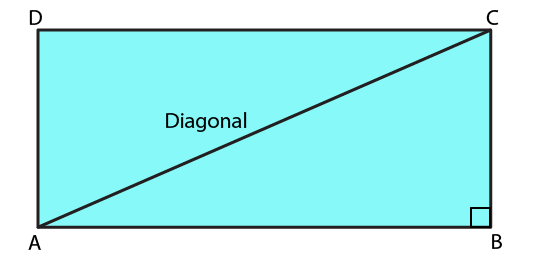AC is the diagonal of rectangle

Consider ABC as a right angled triangle.

AC2 = AB2 + BC2

By substituting the values

640 = 9x2 + x2

We get

640 = 10x2

On further calculation

x2 = 640/10 = 64

x = √64 = 8 m

So the breadth of the rectangle x = 8 m

Length of the rectangle 3x = 3 × 8 = 24 m

Perimeter = 2 (Length + Breadth)

By substituting the values

Perimeter = 2 (24 + 8) = 2 × 32 = 64 m

6. If a diagonal of a rectangle is thrice its smaller side, then its length and breadth are in the ratio
(a) 3: 1
(b) √3: 1
(c) √2 : 1
(d) 2√2: 1

Solution:

The option (d) is the correct answer.

Assume that the length of the smaller side of the rectangle BC = x

Length of the larger side AB = y

It is given that the length of the diagonal is three times that of the smaller side.Diagonal of the rectangle 3x = AC

By using Pythagoras theorem

(AC) 2 = (AB) 2   + (BC) 2

By substituting the values

(3x) 2 = (x) 2 + (y) 2

On further calculation

9x2 = x2 + y2

We get

8x2 = y2

By taking square roots of both sides,

2√2 x = y

Hence, the ratio of the larger side to the smaller side is 2√2: 1.

7. The ratio of the areas of two squares, one having its diagonal double than the other, is
(a) 1: 2
(b) 2: 3
(c) 3: 1
(d) 4: 1

Solution:

The option (d) is the correct answer.

Consider ABCD and PQRS as the two squares. We know that, the diagonal of square PQRS is twice the diagonal of square ABCD.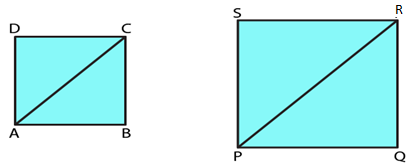PR = 2 AC

Area of the square = Diagonal2/2

Area of PQRS = PR2/2

In the same way, area of ABCD = AC2/2

From the question, we know that:

If AC = x units, we get PR = 2x units

Area of PQRS/Area of ABCD = (PR2×2)/ (2×AC2

By substituting the value

Area of PQRS/Area of ABCD = [(2x) 2×2]/ [2×x2] = 4/1

Hence, the ratio of the areas of squares PQRS and ABCD is 4: 1.

8. If the ratio of areas of two squares is 225 : 256, then the ratio of their perimeters is
(a) 225 : 256
(b) 256 : 225
(c) 15 : 16
(d) 16 : 15

Solution:

The option (c) is the correct answer.

Consider ABCD and PQRS as the two squares.

Let the lengths of each side of ABCD and PQRS be x and y.

We know that

Area of sq. ABCD/Area of sq. PQRS = x2/y2

So we get x2/y2= 225/256

By taking square roots on both sides,

x/y = 15/16

By taking the ratio of their perimeters, we get

Perimeter of sq. ABCD/Primeter of sq. PQRS = (4 × side of sq. ABCD)/ (4 × side of sq. PQRS) = 4x/4y

By removing common terms in numerator and denominator

Perimeter of sq. ABCD/Perimeter of sq. PQRS = x/ y

So perimeter of sq. ABCD/Perimeter of sq. PQRS = 15/16

Hence, the ratio of their perimeters = 15: 16

9. If the sides of a square are halved, then its area
(a) remains same
(b) becomes half
(c) becomes one fourth
(d) becomes double

Solution:

The option (c) is the correct answer.

Consider x as the side of the square.

We know that area of a square = Side × Side = x × x = x2

If the sides are halved, we get new side = x/2

So the new area = (x/2)2= x2/4

From this we know that the area has become one fourth of its previous value.

10. A rectangular carpet has area 120 m2 and perimeter 46 meters. The length of its diagonal is
(a) 15 m
(b) 16 m
(c) 17 m
(d) 20 m

Solution:

The option (c) is the correct answer.

It is given that area of the rectangle = 120 m2

Perimeter of the rectangle = 46 m

Consider l and b as the length and breadth.

Area of the rectangle = l × b = 120 m2

Perimeter of the rectangle = 2 (l + b) = 46

So we get

(l + b) = 46/2 = 23 m

Length of the diagonal of the rectangle = l2 + b2

It can be written as

(l2 + b2) = (l + b) 2 – 2 (l × b)

By substituting the values

(l2 + b2) = (23)2 – 2 (120) = 529 – 240 = 289

By adding square roots on both sides

Length of the diagonal of the rectangle = √l2 + b2 = √289 = 17 m

Hence, the length of the diagonal of the rectangle is 17m.

11. If the ratio between the length and the perimeter of a rectangular plot is 1: 3, then the ratio between the length and breadth of the plot is
(a) 1: 2
(b) 2: 1
(c) 3: 2
(d) 2: 3

Solution:

The option (b) is the correct answer.

Given that Length of rectangle/Perimeter of rectangle = 1/3

So we get

l/ (2l + 2b) = 1/3

By cross multiplication, we get:

3l = 2l + 2b

On further calculation

l = 2b

We get

l/ b= 2/1

Hence, the ratio of the length and the breadth is 2: 1.

12. If the length of the diagonal of a square is 20 cm, then its perimeter is
(a) 10√2cm
(b) 40 cm
(c) 40√2cm
(d) 200 cm

Solution:

The option (c) is the correct answer.

It is given that length of the diagonal = 20 cm

So the length of the side of a square = Length of Diagonal/√2 = 20/√2 = (2 × 10)/√2

We get

Length of the side of a square = (√2 × √2 × 10)/√2 = 10√2 cm

Hence, perimeter of the square = 4 × Side = 4 ×10√2 = 40√2 cm